• 使用py2neo存储将三元组存储到neo4j图形数据库中，构建知识图谱。知䇶图䉡（KQRZOHdJH GUaSK）以结构化的形式描䘠客㿲世界中概念、实体及 其关系，将互㚄㖁的信息㺘䗮成更接䘁人类䇔知世界的形式，提供了一种更好地 ...
• 分类号密级 编号 庫 肀 矸 靛 九 考 硕 士 学位 论 文 中学 课程知识 图谱构 建又 应 用 免 斫 学 申请人姓名 黄 健 位 请 位 生类 全 劊碩士 申 学 学 别 请 位 科 业 申 学 学 专 现代教言 技术学 教 姓 名 名 救 ...
• Python实现了一个简单的知识图谱小demo。非常感谢这篇文章，对这篇文章做了一个复现，并记录遇到的问题。 开发工具：jupyter notebook 开发环境： Python 3.7 en_core_web_sm 2.3.0 spacy 2.2.5 数据集在文章中有...
又是帮着做作业。用Python实现了一个简单的知识图谱小demo。非常感谢这篇文章，对这篇文章做了一个复现，并记录遇到的问题。
开发工具：jupyter notebook
开发环境：
Python 3.7
en_core_web_sm 2.3.0
spacy 2.2.5
数据集在文章中有，自行下载。

# coding: utf-8

# In:

import re
import pandas as pd
import bs4
import requests
import spacy
from spacy import displacy
nlp = spacy.load('en_core_web_sm')

from spacy.matcher import Matcher
from spacy.tokens import Span

import networkx as nx

import matplotlib.pyplot as plt
from tqdm import tqdm

pd.set_option('display.max_colwidth', 200)
get_ipython().run_line_magic('matplotlib', 'inline')

# In:

candidate_sentences = pd.read_csv("wiki_sentences_v2.csv")
candidate_sentences.shape

# In:

candidate_sentences['sentence'].sample(5)

# In:

doc = nlp("the drawdown process is governed by astm standard d823")

for tok in doc:
print(tok.text, "...", tok.dep_)

# In:

def get_entities(sent):
## chunk 1
# 我在这个块中定义了一些空变量。prv tok dep和prv tok text将分别保留句子中前一个单词和前一个单词本身的依赖标签。前缀和修饰符将保存与主题或对象相关的文本。
ent1 = ""
ent2 = ""

prv_tok_dep = "" # dependency tag of previous token in the sentence
prv_tok_text = "" # previous token in the sentence

prefix = ""
modifier = ""

#############################################################

for tok in nlp(sent):
## chunk 2
# 接下来，我们将遍历句子中的记号。我们将首先检查标记是否为标点符号。如果是，那么我们将忽略它并转移到下一个令牌。如果标记是复合单词的一部分(dependency tag = compound)，我们将把它保存在prefix变量中。复合词是由多个单词组成一个具有新含义的单词(例如“Football Stadium”, “animal lover”)。
# 当我们在句子中遇到主语或宾语时，我们会加上这个前缀。我们将对修饰语做同样的事情，例如“nice shirt”, “big house”

# if token is a punctuation mark then move on to the next token
if tok.dep_ != "punct":
# check: token is a compound word or not
if tok.dep_ == "compound":
prefix = tok.text
# if the previous word was also a 'compound' then add the current word to it
if prv_tok_dep == "compound":
prefix = prv_tok_text + " "+ tok.text

# check: token is a modifier or not
if tok.dep_.endswith("mod") == True:
modifier = tok.text
# if the previous word was also a 'compound' then add the current word to it
if prv_tok_dep == "compound":
modifier = prv_tok_text + " "+ tok.text

## chunk 3
# 在这里，如果令牌是主语，那么它将作为ent1变量中的第一个实体被捕获。变量如前缀，修饰符，prv tok dep，和prv tok文本将被重置。
if tok.dep_.find("subj") == True:
ent1 = modifier +" "+ prefix + " "+ tok.text
prefix = ""
modifier = ""
prv_tok_dep = ""
prv_tok_text = ""

## chunk 4
# 在这里，如果令牌是宾语，那么它将被捕获为ent2变量中的第二个实体。变量，如前缀，修饰符，prv tok dep，和prv tok文本将再次被重置。
if tok.dep_.find("obj") == True:
ent2 = modifier +" "+ prefix +" "+ tok.text

## chunk 5
# 一旦我们捕获了句子中的主语和宾语，我们将更新前面的标记和它的依赖标记。
# update variables
prv_tok_dep = tok.dep_
prv_tok_text = tok.text
#############################################################

return [ent1.strip(), ent2.strip()]

get_entities('the film had 200 patents')

# In:

entity_pairs = []

for i in tqdm(candidate_sentences["sentence"]):
entity_pairs.append(get_entities(i))

entity_pairs[10:20]

# In:

def get_relation(sent):

doc = nlp(sent)

# Matcher class object
matcher = Matcher(nlp.vocab)

#define the pattern
pattern = [{'DEP':'ROOT'},
{'DEP':'prep','OP':"?"},
{'DEP':'agent','OP':"?"},
{'POS':'ADJ','OP':"?"}]

matcher.add("matching_1", None, pattern)

matches = matcher(doc)
k = len(matches) - 1

span = doc[matches[k]:matches[k]]

return(span.text)

get_relation("John completed the task")

# In:

relations = [get_relation(i) for i in tqdm(candidate_sentences['sentence'])]

pd.Series(relations).value_counts()[:50]

# In:

# extract subject
source = [i for i in entity_pairs]
# extract object
target = [i for i in entity_pairs]

kg_df = pd.DataFrame({'source':source, 'target':target, 'edge':relations})

# In:

# create a directed-graph from a dataframe
G=nx.from_pandas_edgelist(kg_df, "source", "target", edge_attr=True, create_using=nx.MultiDiGraph())

# In:

plt.figure(figsize=(12,12))

pos = nx.spring_layout(G)
nx.draw(G, with_labels=True, node_color='skyblue', edge_cmap=plt.cm.Blues, pos = pos)
plt.show()

# In:

G=nx.from_pandas_edgelist(kg_df[kg_df['edge']=="composed by"], "source", "target",
edge_attr=True, create_using=nx.MultiDiGraph())

plt.figure(figsize=(12,12))
pos = nx.spring_layout(G, k = 0.5) # k regulates the distance between nodes
nx.draw(G, with_labels=True, node_color='skyblue', node_size=1500, edge_cmap=plt.cm.Blues, pos = pos)
plt.show()

# In:

G=nx.from_pandas_edgelist(kg_df[kg_df['edge']=="written by"], "source", "target",
edge_attr=True, create_using=nx.MultiDiGraph())

plt.figure(figsize=(12,12))
pos = nx.spring_layout(G, k = 0.5)
nx.draw(G, with_labels=True, node_color='skyblue', node_size=1500, edge_cmap=plt.cm.Blues, pos = pos)
plt.show()

# In:

G=nx.from_pandas_edgelist(kg_df[kg_df['edge']=="released in"], "source", "target",
edge_attr=True, create_using=nx.MultiDiGraph())

plt.figure(figsize=(12,12))
pos = nx.spring_layout(G, k = 0.5)
nx.draw(G, with_labels=True, node_color='skyblue', node_size=1500, edge_cmap=plt.cm.Blues, pos = pos)
plt.show()




展开全文• 知识图谱——从零开始用neo4j框架对csv文件构建知识图谱（一）——安装neo4j 知识图谱——从零开始用neo4j框架对csv文件构建知识图谱（二）——构建三元组 1.构建实体文件和关系文件 在上文我们最后得出了entity-...
知识图谱——从零开始用neo4j框架对csv文件构建知识图谱（一）——安装neo4j
知识图谱——从零开始用neo4j框架对csv文件构建知识图谱（二）——构建三元组
1.构建实体文件和关系文件
在上文我们最后得出了entity-relation.csv，接下来我们对其拆分为实体和关系两个文件
#!/usr/bin/env python
# _*_ coding:utf-8 _*_

import pandas as pd
import csv

# 读取三元组文件
h_r_t_name = [":START_ID", "role", ":END_ID"]
h_r_t = pd.read_table("entity_relation.csv", decimal="\t", names=h_r_t_name,delimiter=",")
# print(h_r_t.info())
# print(h_r_t.head())

# 去除重复实体
entity = set()
entity_h = h_r_t[':START_ID'].tolist()
entity_t = h_r_t[':END_ID'].tolist()
for i in entity_h:
entity.add(i)
for i in entity_t:
entity.add(i)
print(entity)
# 保存节点文件
csvf_entity = open("entity.csv", "w", newline='', encoding='utf-8')
w_entity = csv.writer(csvf_entity)
# 实体ID，要求唯一，名称，LABEL标签，可自己不同设定对应的标签
w_entity.writerow(("entity:ID", "name", ":LABEL"))
entity = list(entity)
entity_dict = {}
for i in range(len(entity)):
w_entity.writerow(("e" + str(i), entity[i], "my_entity"))
entity_dict[entity[i]] = "e" + str(i)
csvf_entity.close()
# 生成关系文件，起始实体ID，终点实体ID，要求与实体文件中ID对应，:TYPE即为关系
h_r_t[':START_ID'] = h_r_t[':START_ID'].map(entity_dict)
h_r_t[':END_ID'] = h_r_t[':END_ID'].map(entity_dict)
h_r_t[":TYPE"] = h_r_t['role']
h_r_t.pop('role')
h_r_t.to_csv("roles.csv", index=False)

entity.csv文件如下图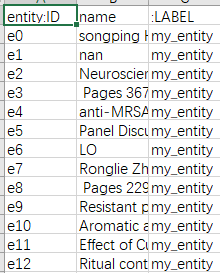roles.csv文件如下图所示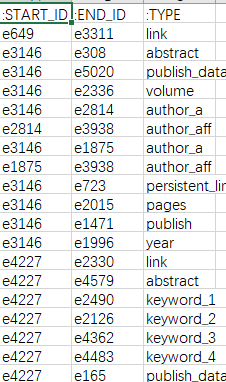2.调用neo4j构建知识图谱
在命令行输入neo4j-admin.bat import --nodes D:\python_workplaces\get_kg\entity.csv --relationships D:\python_workplaces\get_kg\roles.csv（对于换成自己的链接）
成功的话会在data文件夹下的databases文件夹下出现graph.db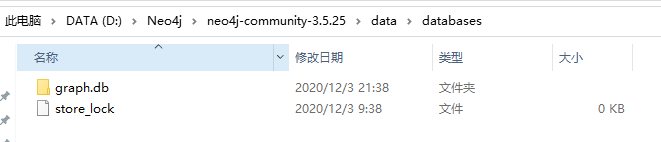这时候重新打开一个新的命令行，输入neo4j.bat console，打开http://localhost:7474/browser/，登陆，左侧点击，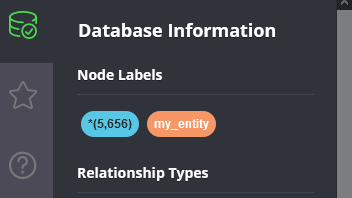即会看到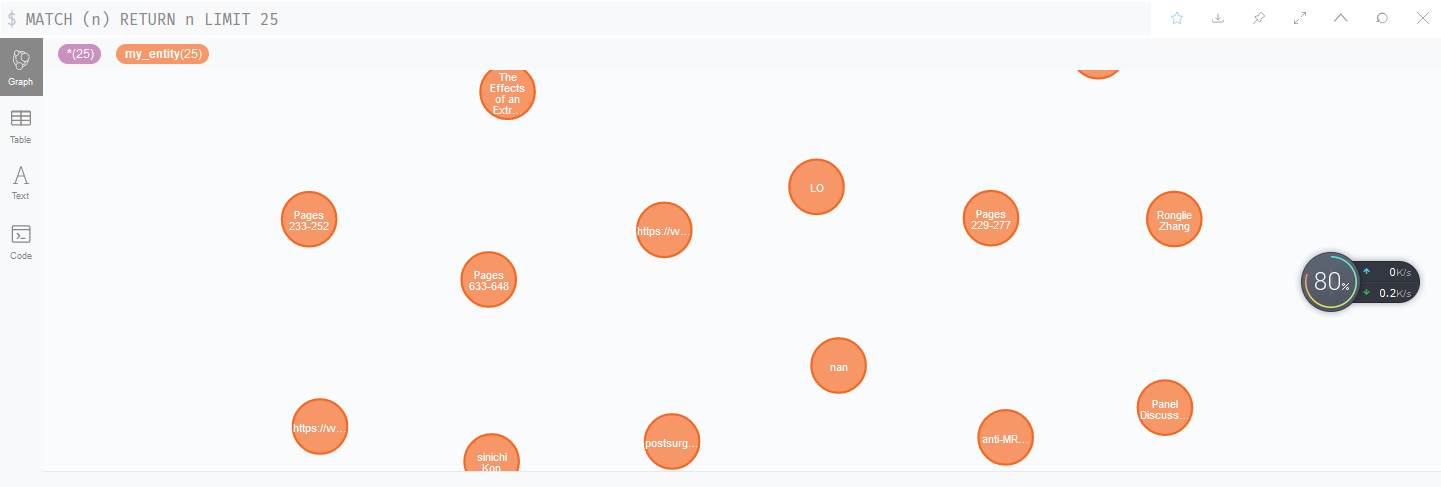说明成功了
3.失败的问题有
（1）实体错误，常见为实体不是一行输入的，是多行，出错信息中出现e222 error字样，修改为一行即可
（2）关系对应上，常见为没有该实体，，出错信息为e222 missing，把出错的实体补上就行，我才用的是把其设置为一个error实体
（3）出现NAN实体，把这个实体及其关系去掉即可，或者一开始就不把nan作为一个实体


展开全文neo4j csv
• 本篇文章主要采用Python和Gephi构建中国知网某个领域的作者合作关系和主题词共现的知识图谱，重点阐述了一种可操作的关系图谱构建方法，可用于论文发表、课程或企业可视化展示等。其基本步骤如下：1.在中国知网搜索...
该系列文章主要讲解知识图谱或关系图谱的构建方法，前文介绍了Neo4j图数据库和Jieba、PyLTP的基本用法。本篇文章主要采用Python和Gephi构建中国知网某个领域的作者合作关系和主题词共现的知识图谱，重点阐述了一种可操作的关系图谱构建方法，可用于论文发表、课程或企业可视化展示等。其基本步骤如下：
1.在中国知网搜索“清水江”关键词，并导出论文Excel格式。
2.使用Python处理文本，获取作者合作的共现矩阵及三元组。
3.Gephi导入CSV节点及边文件，并构建关系图谱。
4.Gephi调整参数，优化关系图谱。
这是一篇非常基础的文章，而且不需要撰写网络爬虫，最终生成的效果还不错，可广泛应用于知识图谱、引文分析、企业合作等领域。希望大家喜欢，尤其是研究自然语言处理和知识图谱的同学。同时，从今天起，博客的代码尽量上传到Github和CSDN下载，脚踏实地，与君同在。
下载地址：https://download.csdn.net/download/eastmount/11650953
文章目录一.最终效果图二.中国知网导出数据三.什么是共现关系四.Python构建共现矩阵和三元组五.Gephi构建知识图谱(一).数据准备(二).数据导入(三).知识图谱参数设置(四).可视化显示六.总结
前文参考
Neo4j图数据库系列：
[知识图谱构建] 一.Neo4j图数据库安装初识及药材供应图谱实例
[知识图谱构建] 二.《Neo4j基础入门》基础学习之创建图数据库节点及关系
[知识图谱构建] 三.Neo4j创建高校教师关系图谱及查询语句入门详解
Python知识图谱构建系列：
[Python知识图谱] 一.哈工大pyltp安装及中文分句、中文分词、导入词典基本用法
[Python知识图谱] 二.哈工大pyltp词性标注、命名实体识别、依存句法分析和语义角色标注
[Python知识图谱] 三.Jieba工具中文分词、添加自定义词典及词性标注详解
关系图谱系列：
[关系图谱] 一.Gephi通过共现矩阵构建知网作者关系图谱
[关系图谱] 二.Gephi导入共线矩阵构建作者关系图谱
知识图谱实例系列：
[知识图谱实战篇] 一.数据抓取之Python3抓取JSON格式的电影实体
[知识图谱实战篇] 二.Json+Seaborn可视化展示电影实体
[知识图谱实战篇] 三.Python提取JSON数据、HTML+D3构建基本可视化布局
[知识图谱实战篇] 四.HTML+D3+CSS绘制关系图谱
[知识图谱实战篇] 五.HTML+D3添加鼠标响应事件显示相关节点及边
[知识图谱实战篇] 六.HTML+D3实现点击节点显示相关属性及属性值
[知识图谱实战篇] 七.HTML+D3实现关系图谱搜索功能
[知识图谱实战篇] 八.HTML+D3绘制时间轴线及显示实体

一.最终效果图
作者合作知识图谱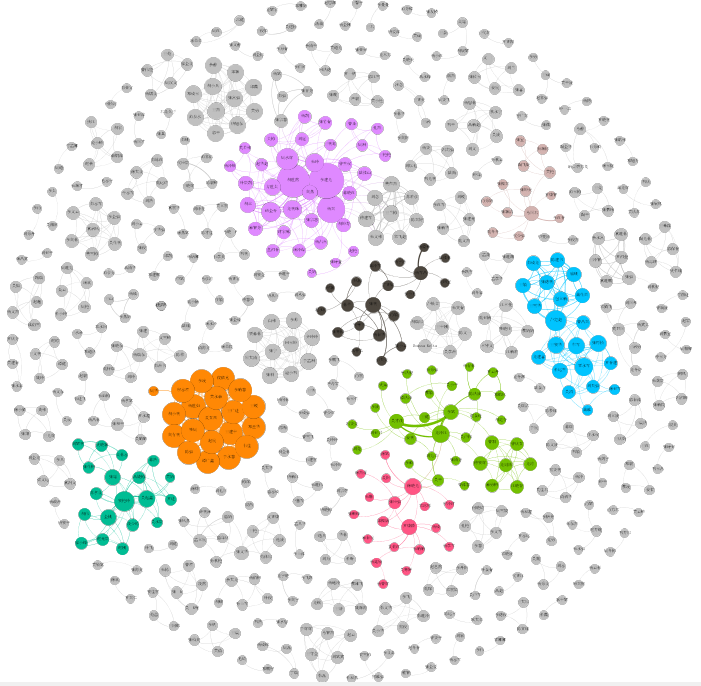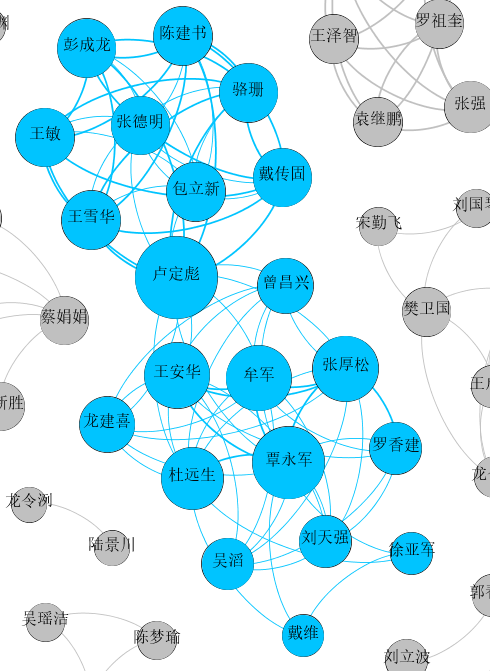下面是另一个主题“网络安全”以某作者为中心的区域，分别是武大和北邮。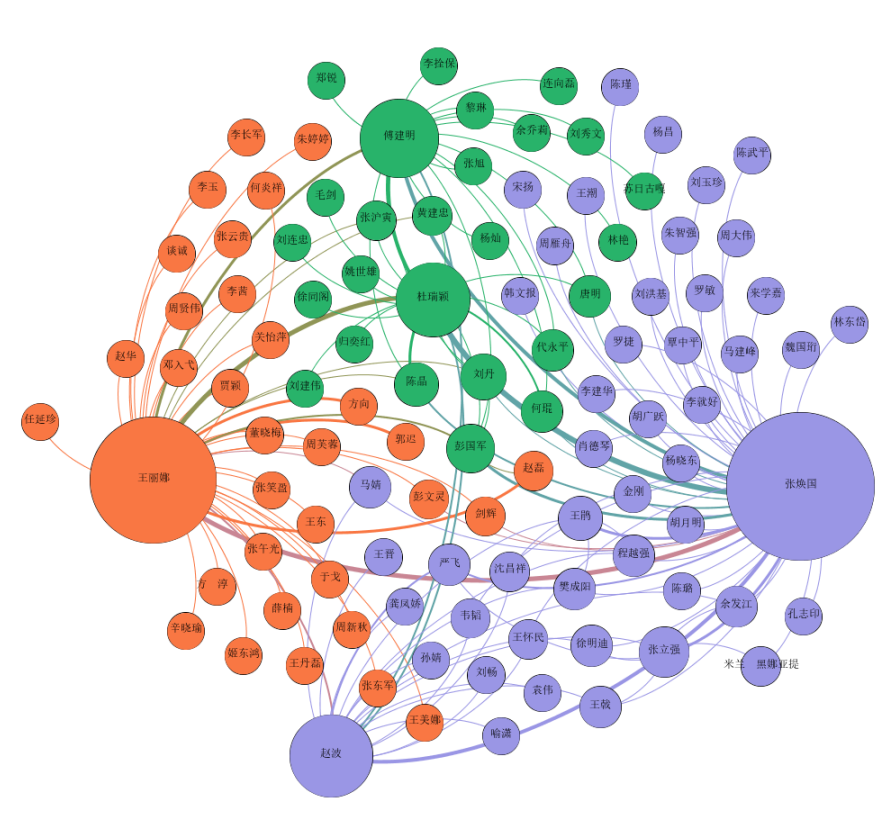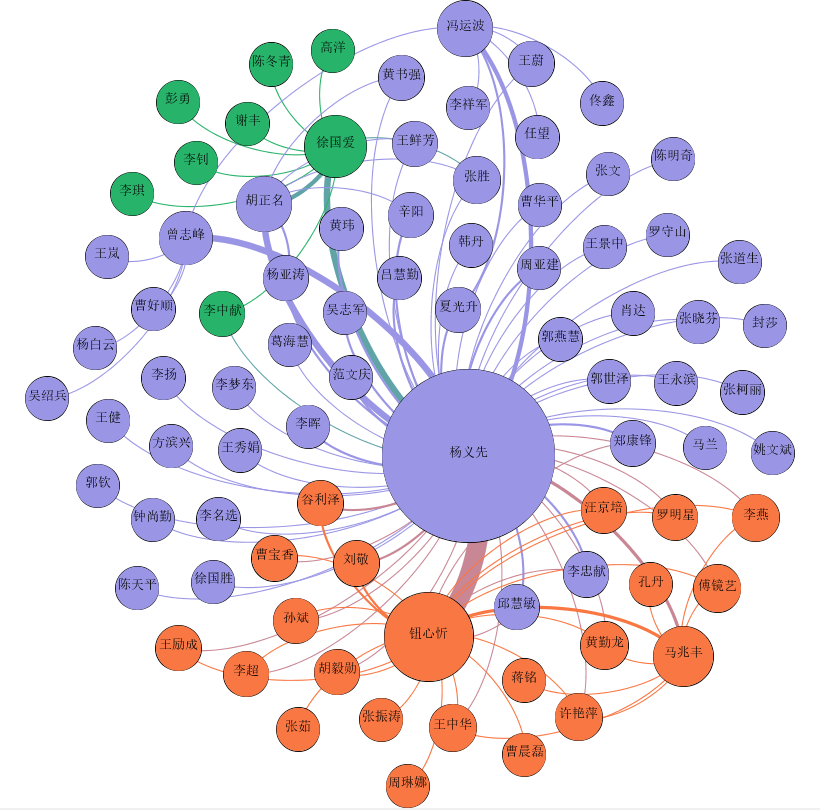关键词共现知识图谱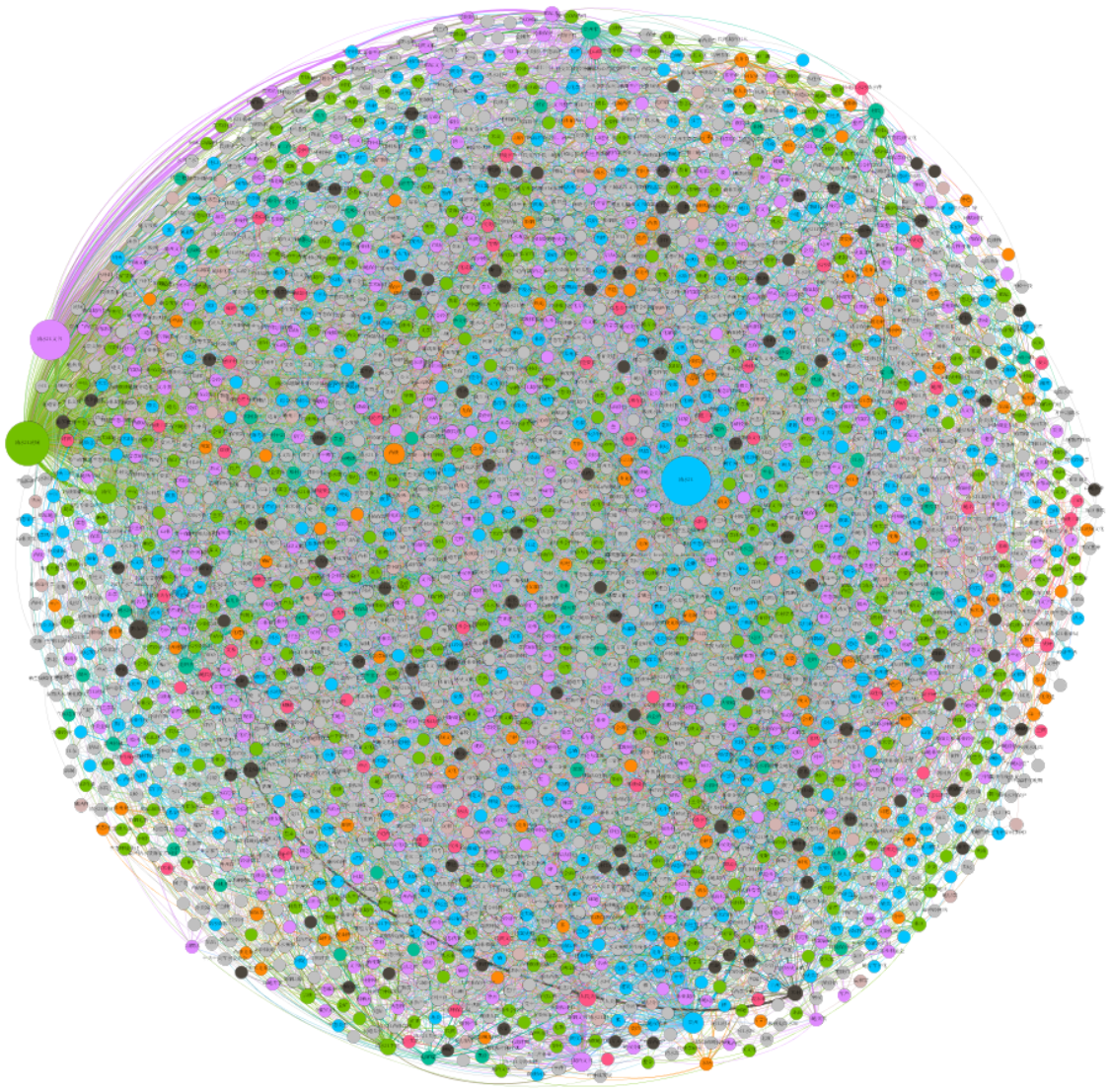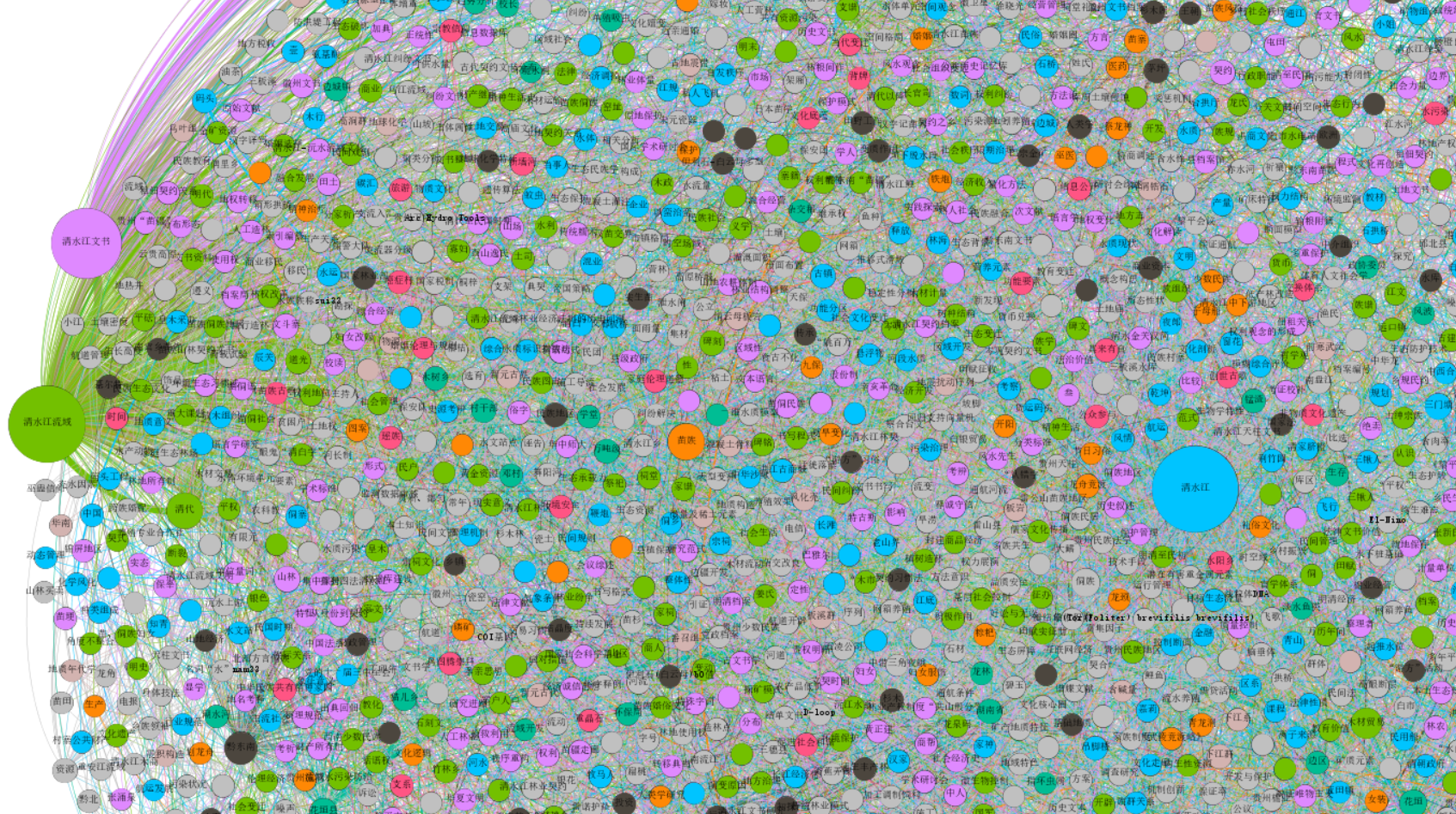二.中国知网导出数据
第一步：在中国知网CNKI中搜索“清水江”关键词，返回期刊论文1007篇，作为目标分析数据。
注意，中国知网最多能导出5000篇文献，如果文章过多建议增加按年份统计，最后再汇总。同时，由于博士论文、会议论文、报纸作者关系较为单一，所以这里仅分析期刊论文。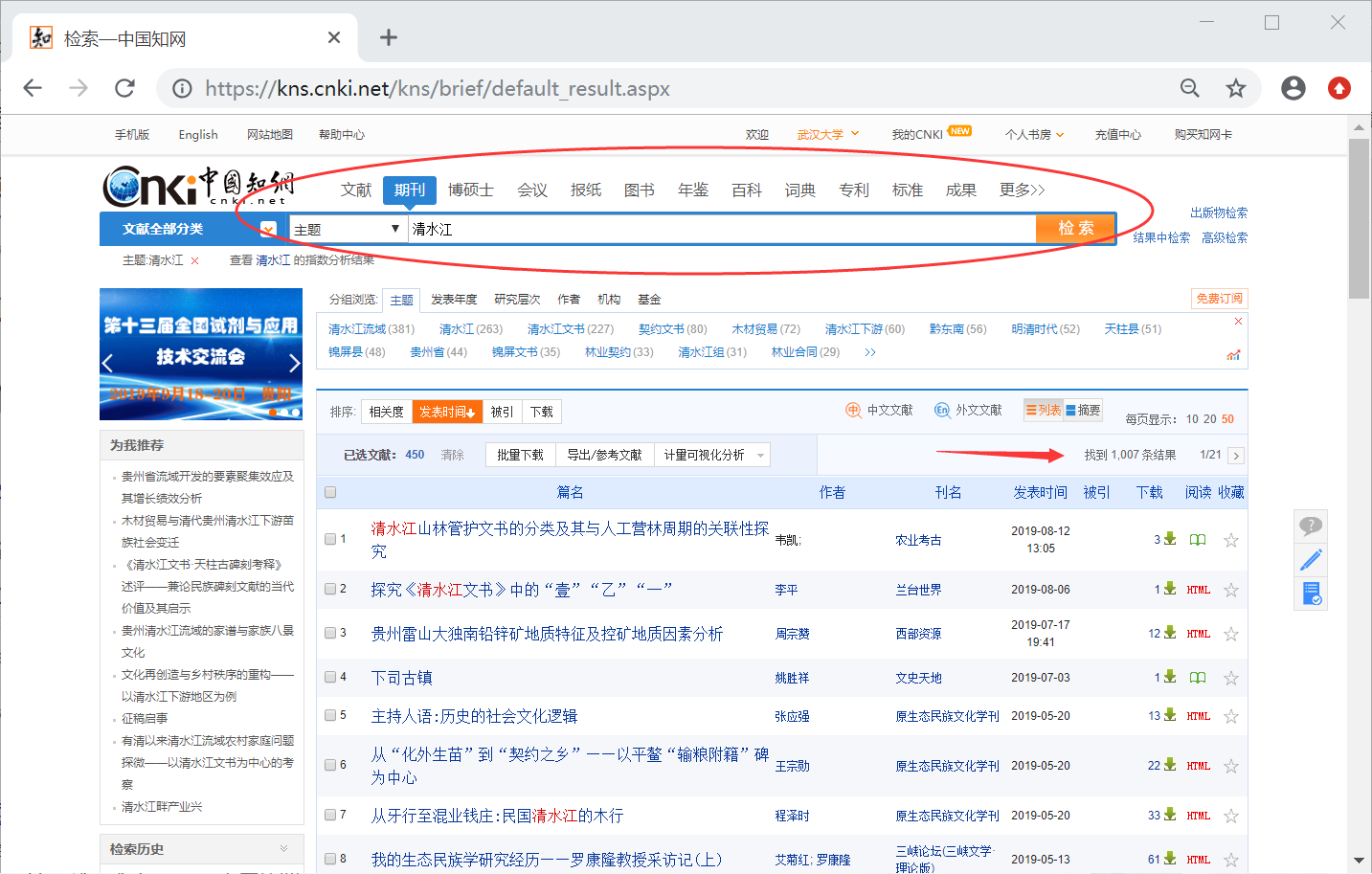第二步：点击左上角勾选文献。每次最多选择50篇论文，接着点击下一页，导出500篇文献。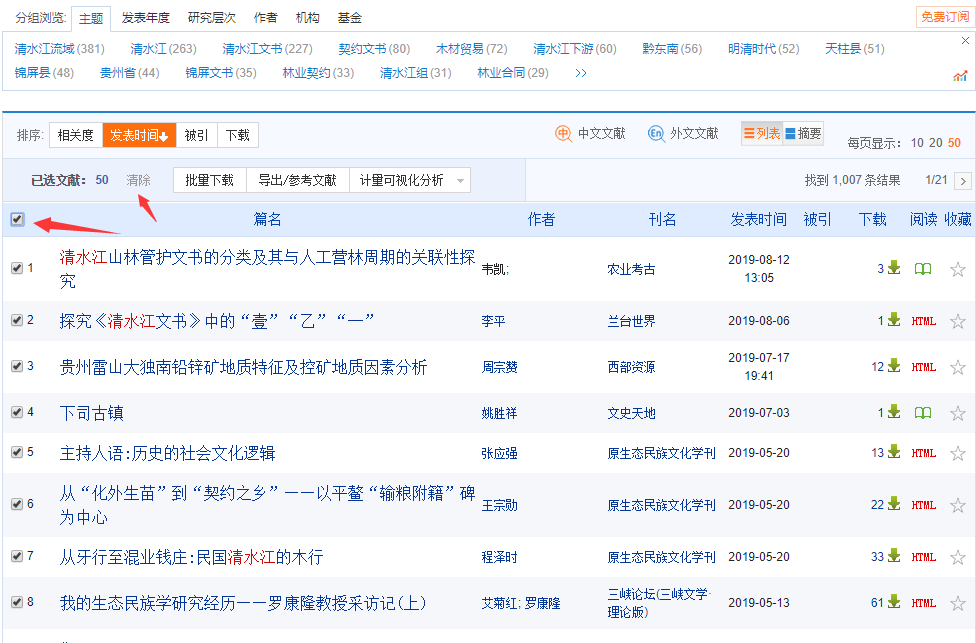选择下一页：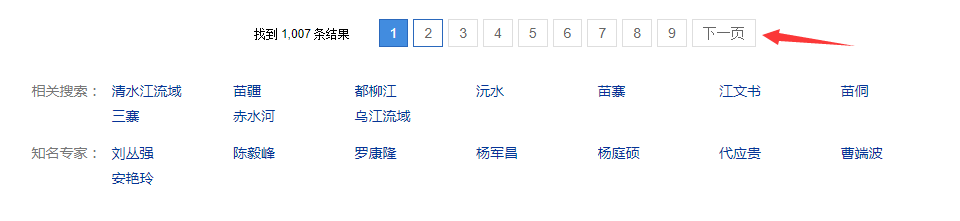选中500篇论文之后，接着点击“导出/参考文献”选项。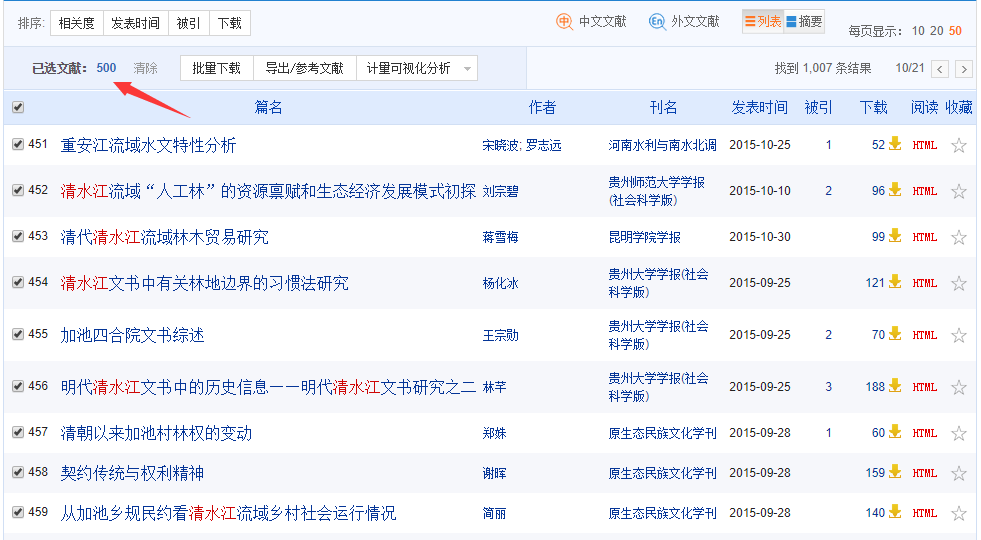第三步：在弹出的 界面中，选择“Reforks”格式，点击XLS导出。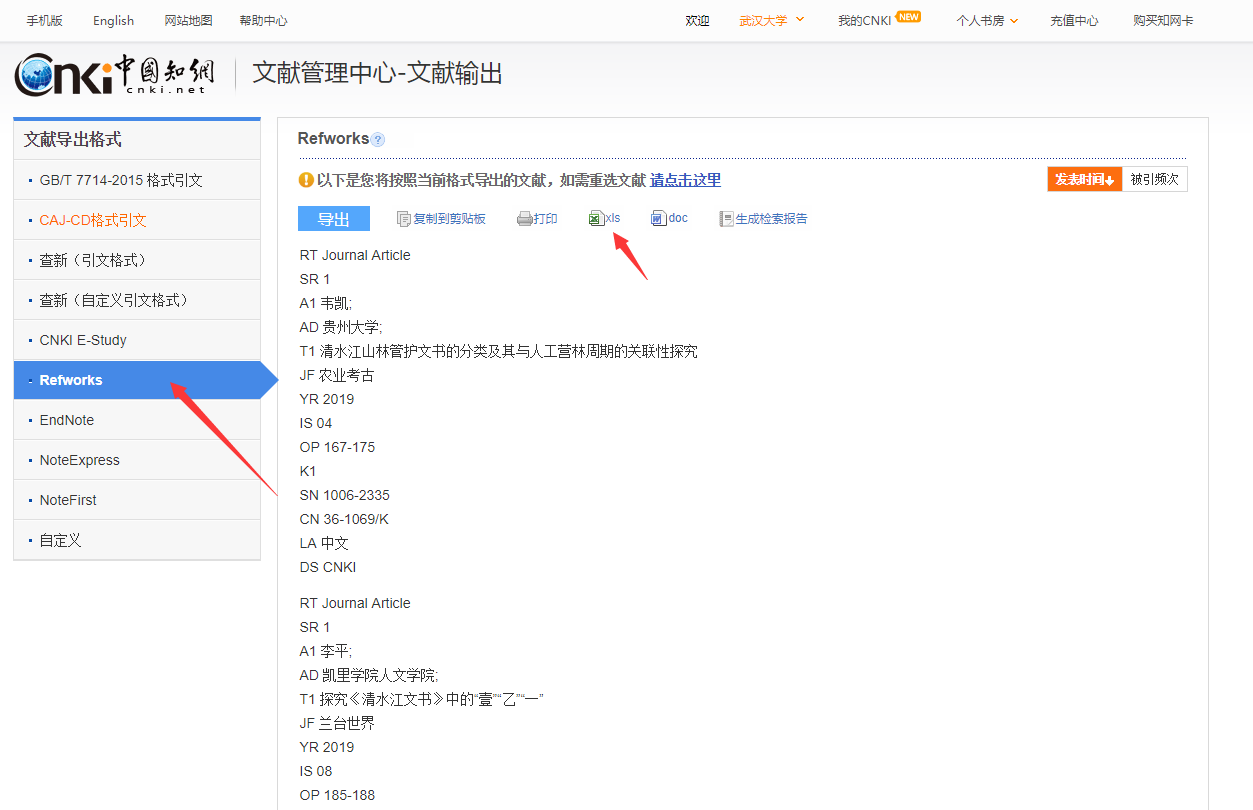到处的表格如下图所示，包括各种论文信息，可惜没有下载量和被引用量（网络爬虫实现），但我们的知识图谱分析也够了。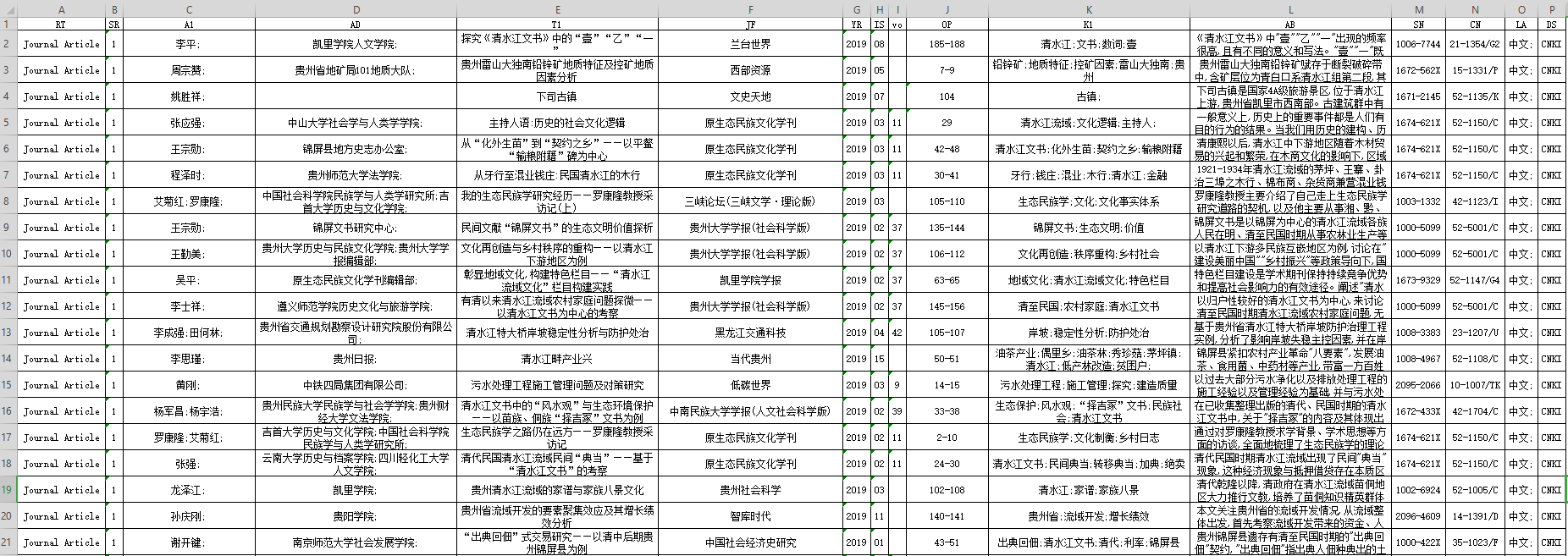第四步：再下载剩余的所有文献，每次500篇的下载，并整理在一个Excel表格中，过滤掉英文和无作者的论文，剩下958篇，即为分析的数据集。三.什么是共现关系
引文分析常见的包括两类，一种是共现关系，另一种是引用和被引用关系。本文主要讲解共现关系，假设现在存在四篇文章，如下所示：
文章标题                                        作者
大数据发展现状分析                              A，B，C，D
Python网络爬虫                                 A，B，C
贵州省大数据战略                                 B，A，D
大数据分析重要                                    D，A

第一步：写代码抓取该领域文章的所有作者，即：A、B、C、D。
第二步：接着获取对应的共现矩阵，比如文章“大数据发展现状分析”，则认为A、B、C、D共现，在他们之间建立一条边。共现矩阵如下所示，共13条边。
$\left[ \begin{matrix} -& A & B & C & D \\ A & 0 & 2 & 2 & 2 \\ B & 1 & 0 & 2 & 2 \\ C & 0 & 0 & 0 & 1 \\ D & 1 & 0 & 0 & 0 \end{matrix} \right] \tag{1}$
第三步：由于最后的图谱是无向图，为了方便计算，我们将矩阵的下三角数据加至上三角，从而减少计算量。
$\left[ \begin{matrix} -& A & B & C & D \\ A & 0 & 3 & 2 & 3 \\ B & 0 & 0 & 2 & 2 \\ C & 0 & 0 & 0 & 1 \\ D & 0 & 0 & 0 & 0 \end{matrix} \right] \tag{1}$
第四步：通过共现矩阵分别获取两两关系及权重，再写入CSV或Excel文件中，如下所示。知识图谱通常由三元组构成，包括 {实体，属性，属性值}、{实体、关系、实体}，这里的{Source、Weight、Weight}也可以称为一个三元组。
$\left[ \begin{matrix} Source & Target & Weight \\ A & B & 3 \\ A & C & 2 \\ A & D & 3 \\ B & C & 2 \\ B & D & 2 \\ C & D & 1 \end{matrix} \right] \tag{2}$
第五步：将该CSV文件导入Gephi中，并绘制相关的图形。因为该实例节点比较少，下面是Pyhton调用Networkx绘制的代码及图形。
# -*- coding: utf-8 -*-
import networkx as nx
import matplotlib.pyplot as plt

#定义有向图
DG = nx.Graph()
#添加五个节点(列表)
DG.add_nodes_from(['A', 'B', 'C', 'D'])
print DG.nodes()
#添加边(列表)
DG.add_edge('A', 'B', weight=3)
DG.add_edge('A', 'C', weight=2)
DG.add_edge('A', 'D', weight=3)
DG.add_edge('B', 'C', weight=2)
DG.add_edge('B', 'D', weight=2)
DG.add_edge('C', 'D', weight=1)
#DG.add_edges_from([('A', 'B'), ('A', 'C'), ('A', 'D'), ('B','C'),('B','D'),('C','D')])
print DG.edges()
#绘制图形 设置节点名显示\节点大小\节点颜色
colors = ['red', 'green', 'blue', 'yellow']
nx.draw(DG,with_labels=True, node_size=900, node_color = colors)
plt.show()

绘制图形如下所示：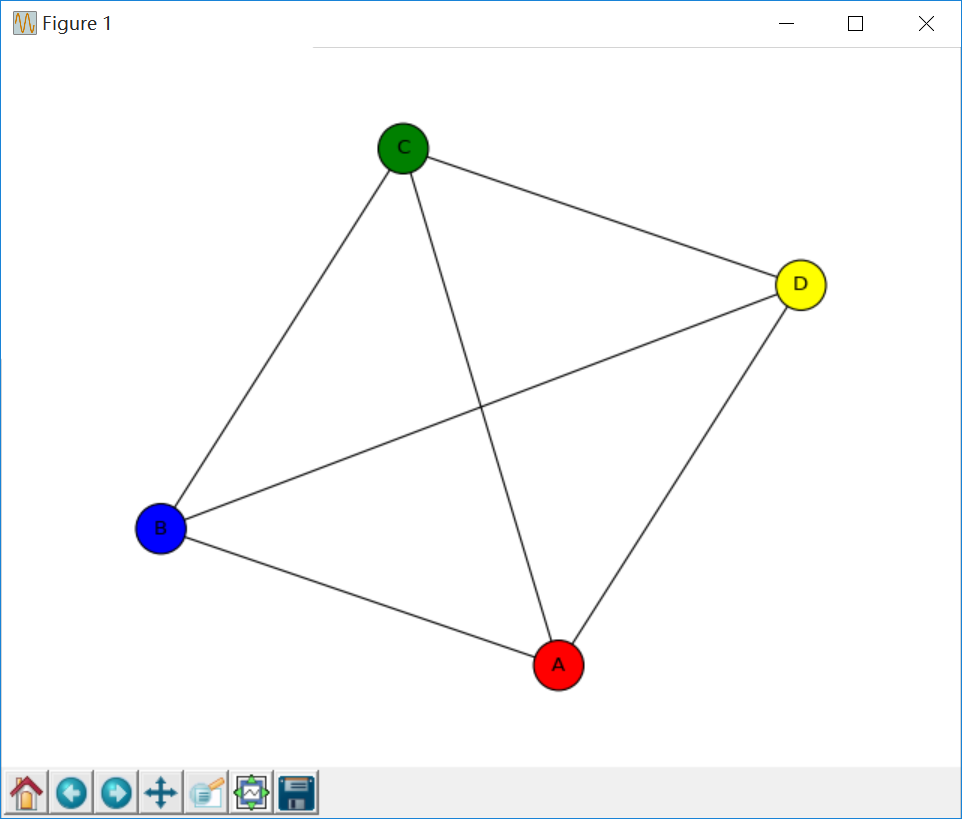接下来我们将告诉大家如何撰写Python代码获取作者共现矩阵及三元组。

四.Python构建共现矩阵和三元组
第一步 ：将Excel中作者列单独提取至word.txt文件中，如下图所示。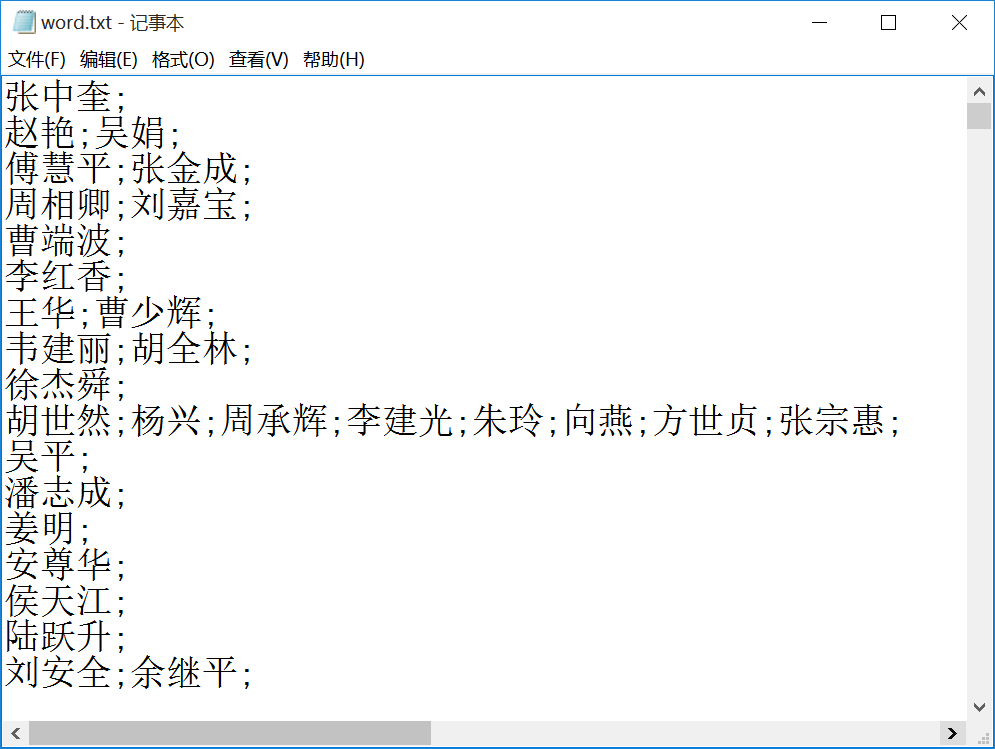第二步：运行Python代码获取共现矩阵和{Source， Weight，Target}三元组。

按行读取TXT数据，并调用split(’;’)分割作者，将所有作者信息存储至word列表中（不含重复项）
建立 word_vector[作者数][作者数] 共现矩阵，依次获取每行的两个作者下标位置，记录在变量w1、w2、n1、n2中
词频矩阵赋值，这里通过判断计算上三角矩阵 word_vector[n1][n2] += 1 或 word_vector[n2][n1] += 1
两层循环获取共现矩阵 word_vector[i][j] 中不为0的数值，word[i]、word[j] 和权重 word_vector[i][j] 即为三元组
将最终结果输出至TXT和CSV文件

# -*- coding: utf-8 -*-
"""
@author: eastmount CSDN 杨秀璋 2019-09-02
"""
import pandas as pd
import numpy as np
import codecs
import networkx as nx
import matplotlib.pyplot as plt
import csv

#---------------------------第一步:读取数据-------------------------------
word = [] #记录关键词
f = open("word.txt")
line = f.readline()
while line:
#print line
line = line.replace("\n", "") #过滤换行
line = line.strip('\n')
for n in line.split(';'):
#print n
if n not in word:
word.append(n)
line = f.readline()
f.close()
print len(word) #作者总数

#--------------------------第二步 计算共现矩阵----------------------------
a = np.zeros([2,3])
print a

#共现矩阵
#word_vector = np.zeros([len(word),len(word)], dtype='float16')

#MemoryError：矩阵过大汇报内存错误
#https://jingyan.baidu.com/article/a65957f434970a24e67f9be6.html
#采用coo_matrix函数解决该问题

from scipy.sparse import coo_matrix
print len(word)
#类型<type 'numpy.ndarray'>
word_vector = coo_matrix((len(word),len(word)), dtype=np.int8).toarray()
print word_vector.shape

f = open("word.txt")
line = f.readline()
while line:
line = line.replace("\n", "") #过滤换行
line = line.strip('\n') #过滤换行
nums = line.split(';')

#循环遍历关键词所在位置 设置word_vector计数
i = 0
j = 0
while i<len(nums):       #ABCD共现 AB AC AD BC BD CD加1
j = i + 1
w1 = nums[i]           #第一个单词
while j<len(nums):
w2 = nums[j]       #第二个单词
#从word数组中找到单词对应的下标
k = 0
n1 = 0
while k<len(word):
if w1==word[k]:
n1 = k
break
k = k +1
#寻找第二个关键字位置
k = 0
n2 = 0
while k<len(word):
if w2==word[k]:
n2 = k
break
k = k +1
#重点: 词频矩阵赋值 只计算上三角
if n1<=n2:
word_vector[n1][n2] = word_vector[n1][n2] + 1
else:
word_vector[n2][n1] = word_vector[n2][n1] + 1
#print n1, n2, w1, w2
j = j + 1
i = i + 1
#读取新内容
line = f.readline()
f.close()

#--------------------------第三步  TXT文件写入--------------------------
res = open("word_word_weight.txt", "a+")
i = 0
while i<len(word):
w1 = word[i]
j = 0
while j<len(word):
w2 = word[j]
#判断两个词是否共现 共现&词频不为0的写入文件
if word_vector[i][j]>0:
#print w1 +" " + w2 + " "+ str(int(word_vector[i][j]))
res.write(w1 +" " + w2 + " "+ str(int(word_vector[i][j]))  +  "\r\n")
j = j + 1
i = i + 1
res.close()

#共现矩阵写入文件 如果作者数量较多, 建议删除下面部分代码
res = open("word_jz.txt", "a+")
i = 0
while i<len(word):
j = 0
jz = ""
while j<len(word):
jz = jz + str(int(word_vector[i][j])) + " "
j = j + 1
res.write(jz + "\r\n")
i = i + 1
res.close()

#--------------------------第四步  CSV文件写入--------------------------
c = open("word-word-weight.csv","wb")    #创建文件
#c.write(codecs.BOM_UTF8)                   #防止乱码
writer = csv.writer(c)                                #写入对象
writer.writerow(['Word1', 'Word2', 'Weight'])

i = 0
while i<len(word):
w1 = word[i]
j = 0
while j<len(word):
w2 = word[j]
#判断两个词是否共现 共现词频不为0的写入文件
if word_vector[i][j]>0:
#写入文件
templist = []
templist.append(w1)
templist.append(w2)
templist.append(str(int(word_vector[i][j])))
#print templist
writer.writerow(templist)
j = j + 1
i = i + 1
c.close()

如果输入数据为：
A;B;C;D
A;B;C
B;A;D
D;A

则输出结果如下图所示：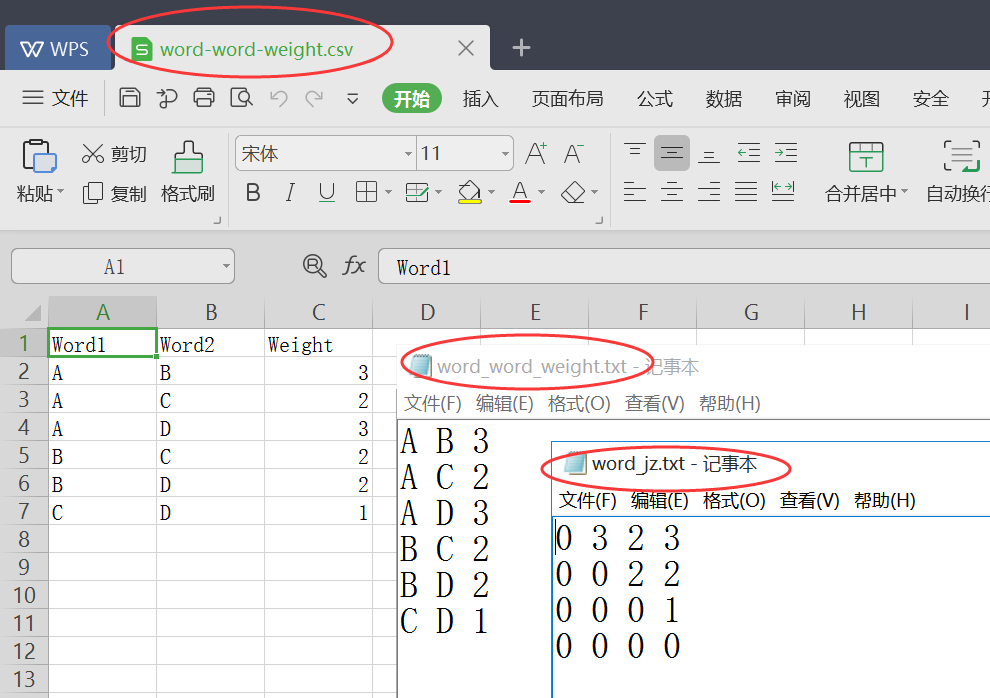第三步：最终的“清水江”文献输出结果如下所示，主要是 word-word-weight.csv 文件。
由于split函数分割过程中，可能存在多余空格的现象，如下图所示，我们将含有空白的栏目删除。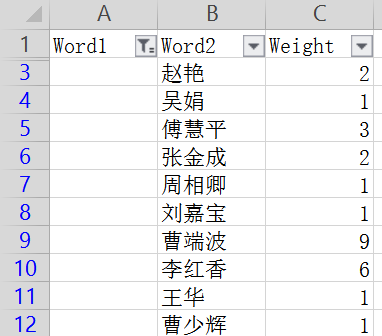完整的三元组共1255组，即构成了1255条边。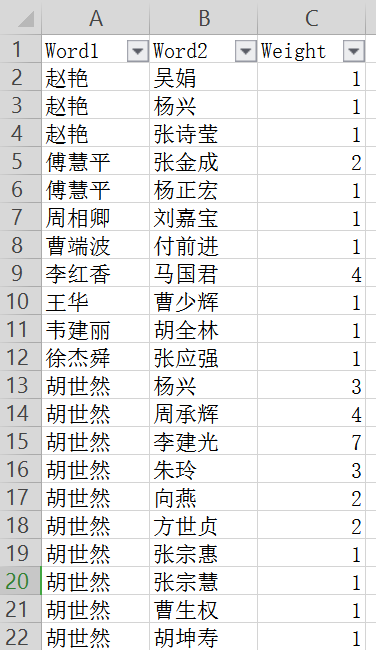注意，如果作者数较多时，代码中尽量不要使用print输出中间结果，并且只做 word-word-weight.csv 文件写入操作即可。同时，建议先尝试运行小的数据集，如前面的ABCD，代码能正确运行之后再加载大的数据集。

五.Gephi构建知识图谱
众所周知，知识图谱都是有节点和边组成的，每一个作者或每一个关键词可以作为一个节点，而共现关系可以构造边。如果共现的次数越多，表示合作越紧密，则边越粗，反之越细。当使用度来表示节点大小时，如果某个节点越大，则表示该节点共现合作的数量越多。下面开始分享Gephi软件绘制关系图谱的流程。
(一).数据准备
edges.csv
包含边的文件就是前面的 word-word-weight.csv，但需要修改表头并增加一列类型Type，其值为Undirected（无向图）。
Source,Target,Type,Weight
赵艳,吴娟,Undirected,1
赵艳,杨兴,Undirected,1
赵艳,张诗莹,Undirected,1
傅慧平,张金成,Undirected,2
傅慧平,杨正宏,Undirected,1
周相卿,刘嘉宝,Undirected,1
曹端波,付前进,Undirected,1
李红香,马国君,Undirected,4
胡世然,杨兴,Undirected,3
胡世然,周承辉,Undirected,4
胡世然,李建光,Undirected,7
胡世然,朱玲,Undirected,3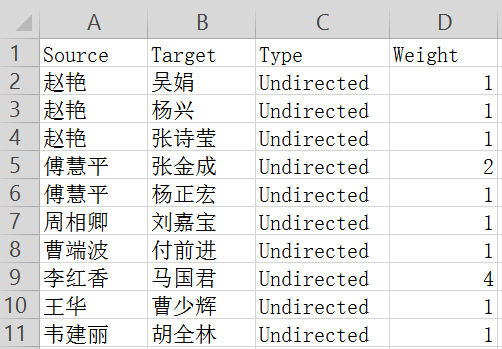nodes.csv
接下来我们还要创建一个包含节点的信息。只需要将Source和Target两列数据复制到nodes.csv中，然后去除重复的即可。当然，如果数量很大，Python写个循环代码也能处理。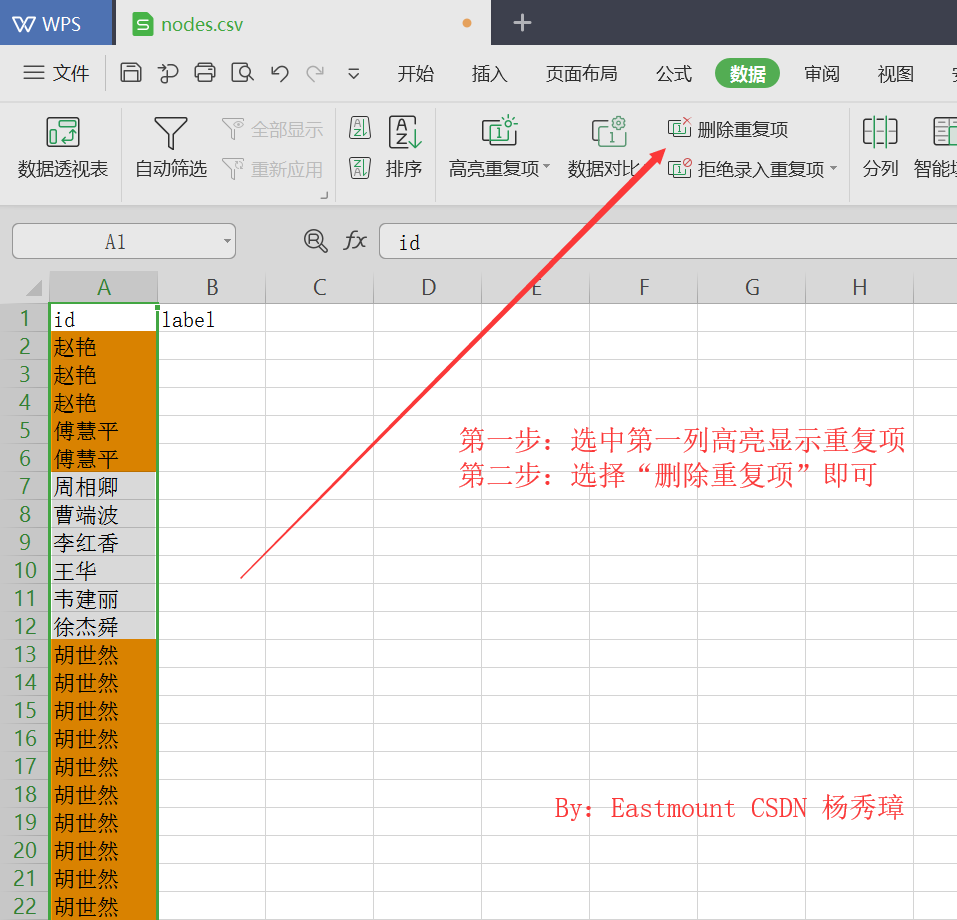接着将第一列复制到第二列，需要注意id表示编号（名称）、label表示节点类标，这里中文显示。nodes.csv最终输出结果如下图所示：
id,label
赵艳,赵艳
傅慧平,傅慧平
周相卿,周相卿
曹端波,曹端波
李红香,李红香
王华,王华
韦建丽,韦建丽
徐杰舜,徐杰舜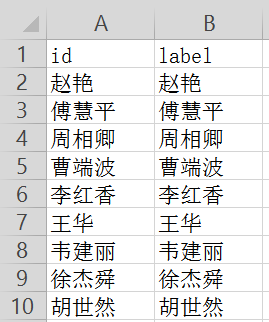(二).数据导入
数据准备充分之后，开始我们的知识图谱构建之旅吧！
第一步：安装并打开Gephi软件。软件我已经上传到下载包中供大家使用了。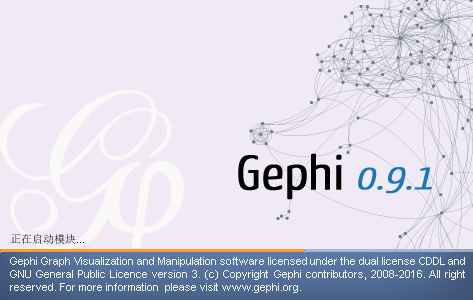第二步：新建工程，并选择“数据资料”，输入电子表格。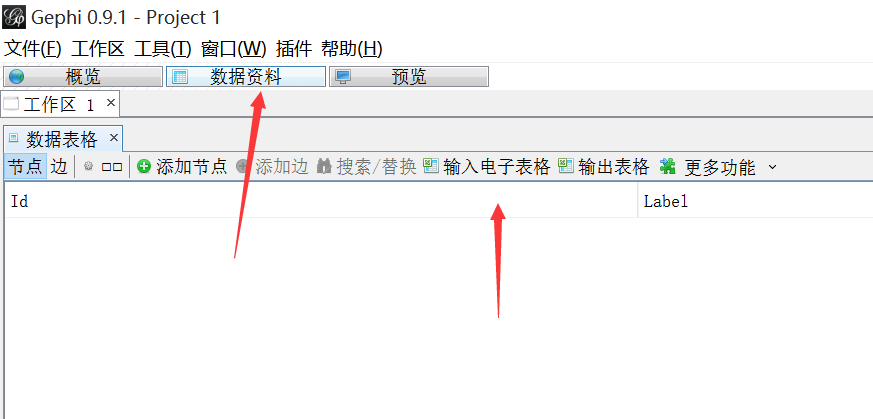第三步：导入节点文件nodes.csv，注意CSV文件中表头切记定位为 id、label，导入一定选择“节点表格”和“GB2312”编码，点击“完成”即可。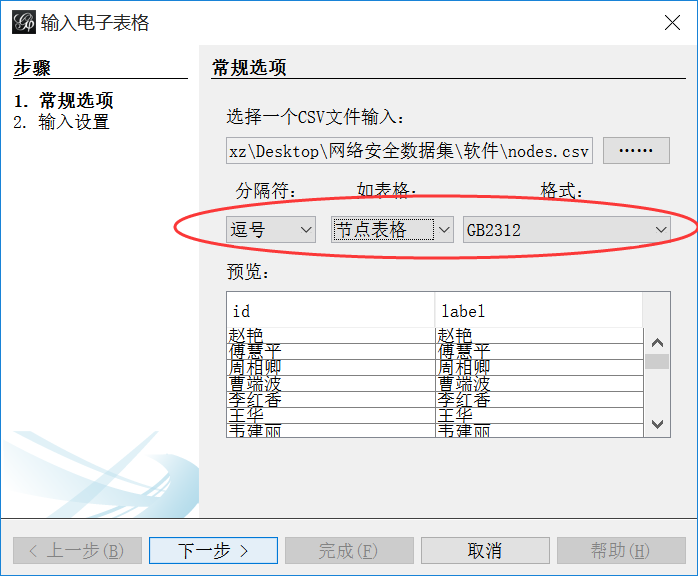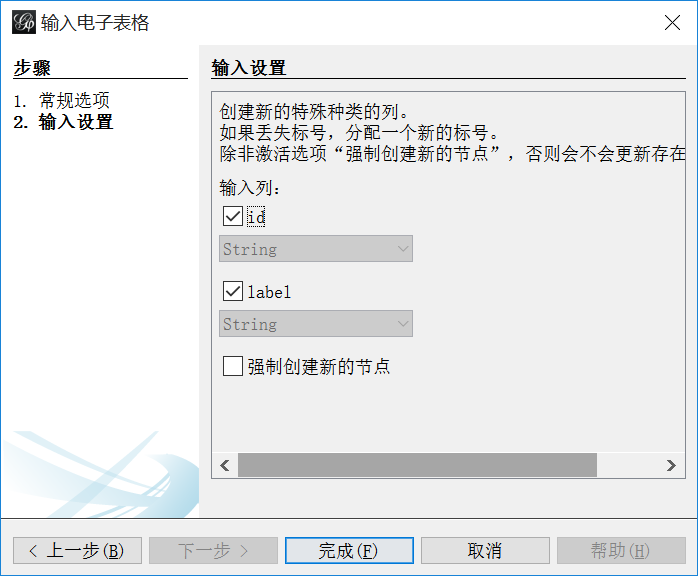第四步：导入边文件edges.csv，注意CSV文件中表头切记为Source（起始点）、Target（目标点）、Weight（权重）、Tpye（无向边 Undirected）。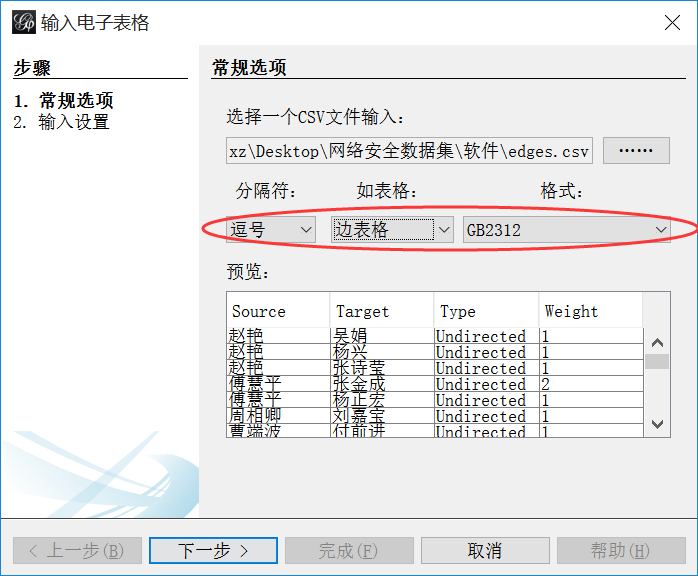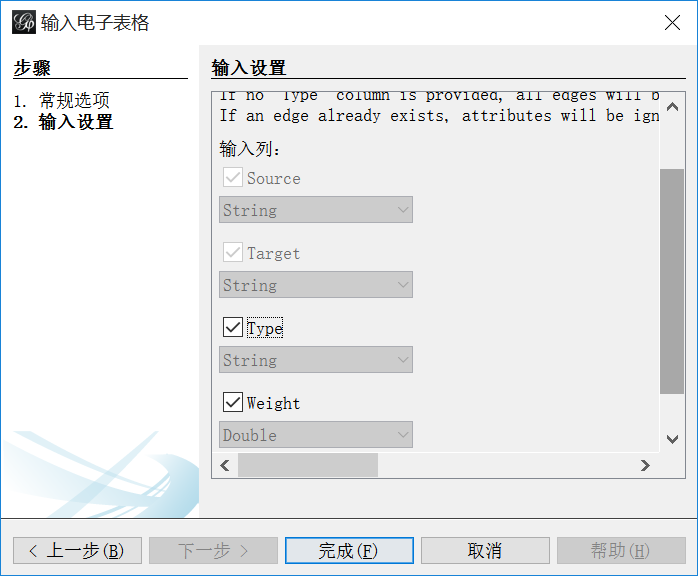导入成功后如下图所示：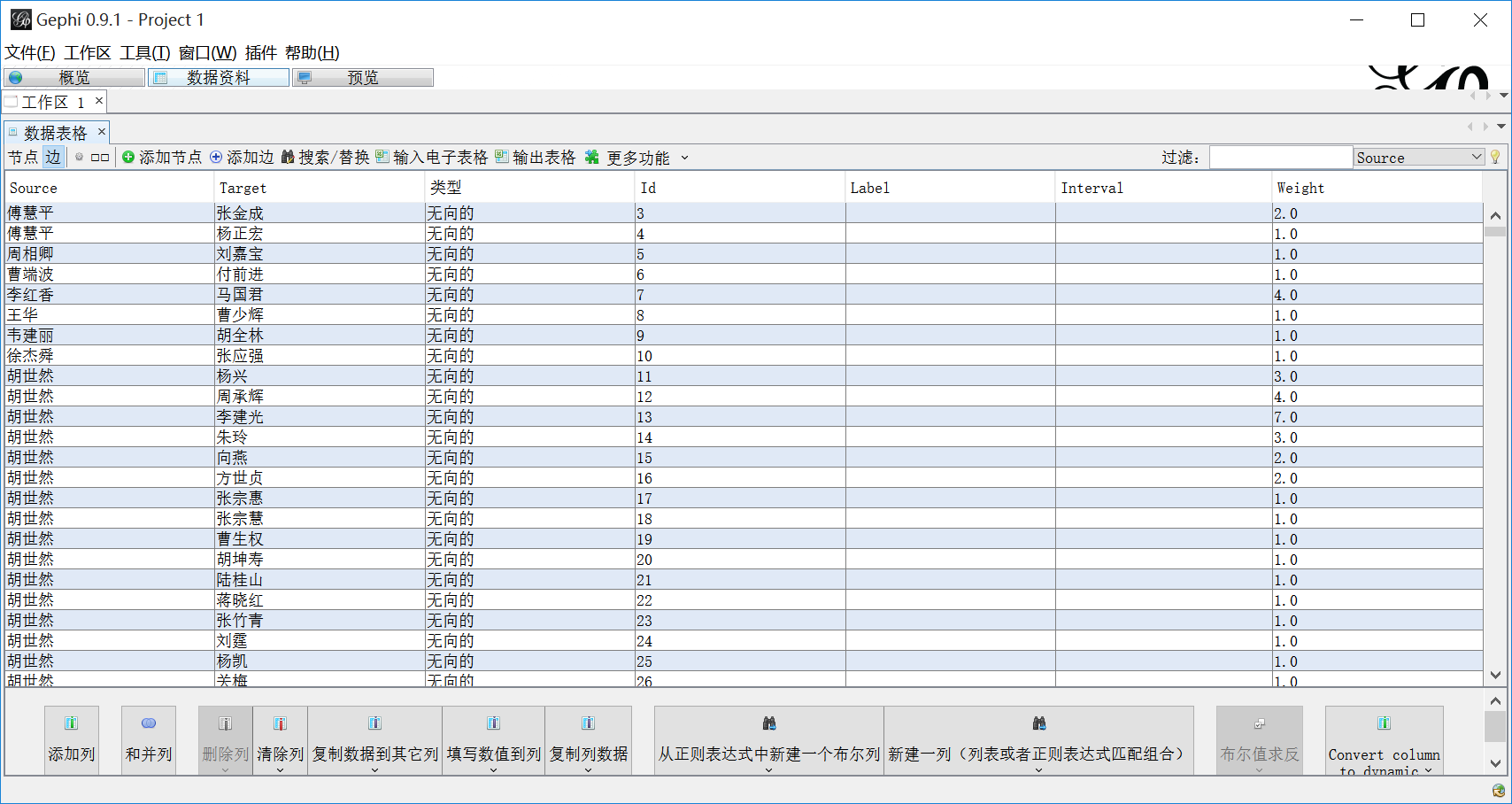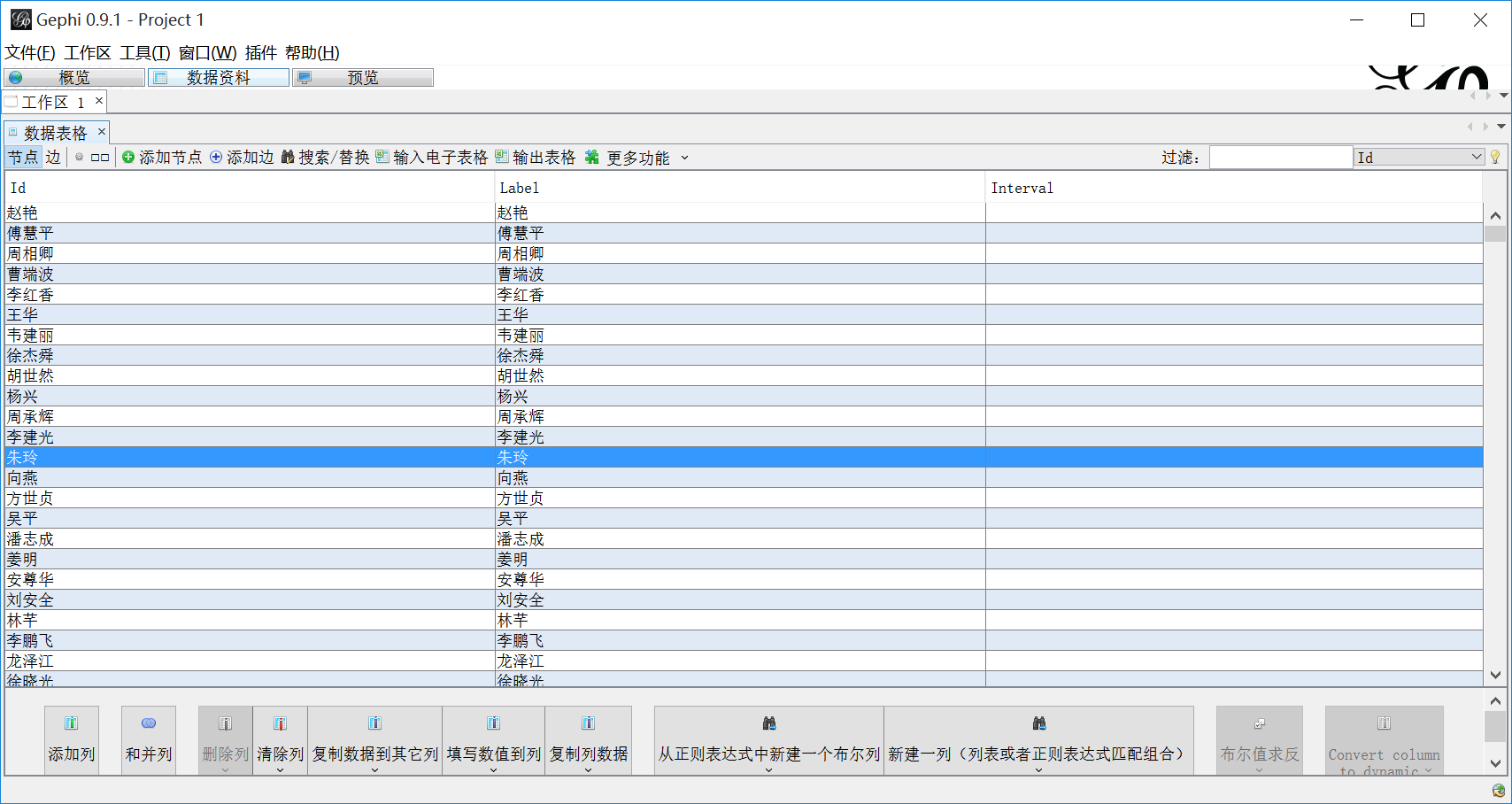导入成功后点击“概览”显示如下所示，接着就是调整参数。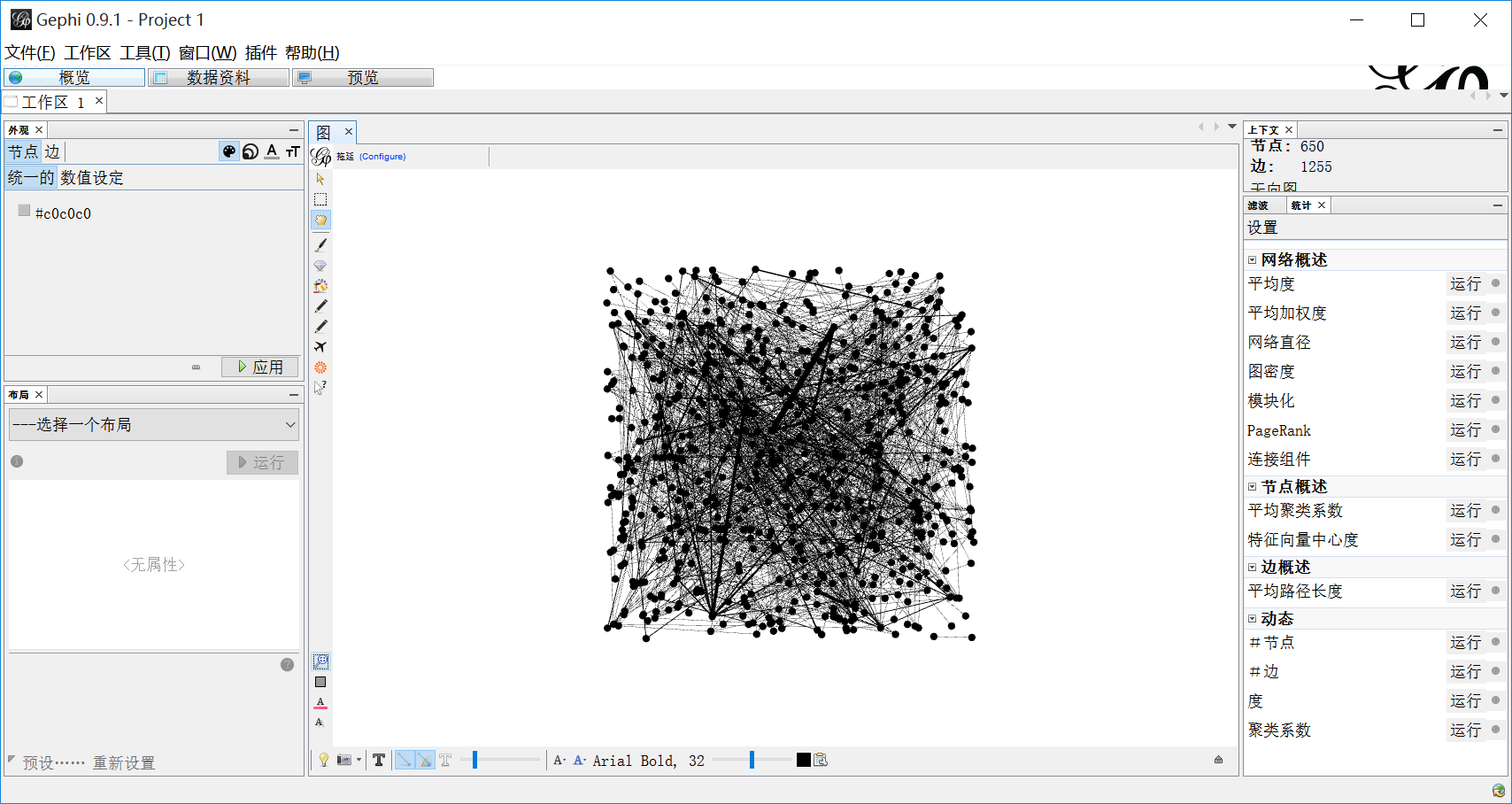(三).知识图谱参数设置
设置外观如下图所示，主要包括颜色、大小、标签颜色、标签尺寸。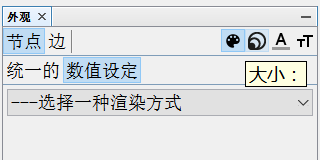1.设置节点大小和颜色。
选中颜色，点击“数值设定”，选择渲染方式为“度”。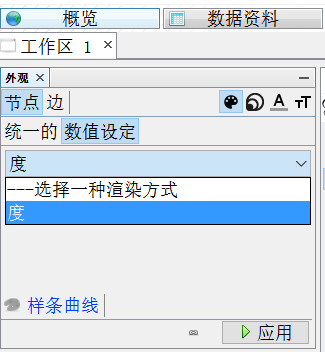显示结果如下所示：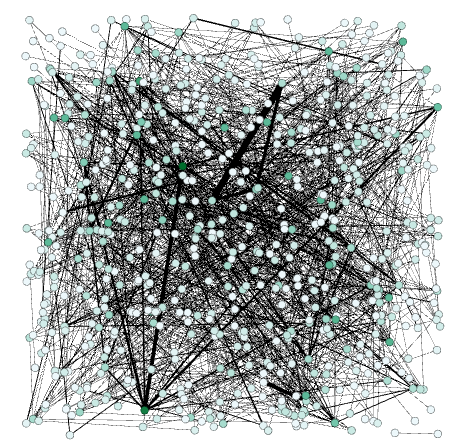接着设置节点大小，数值设定为“度”，最小尺寸为20，最大尺寸为80。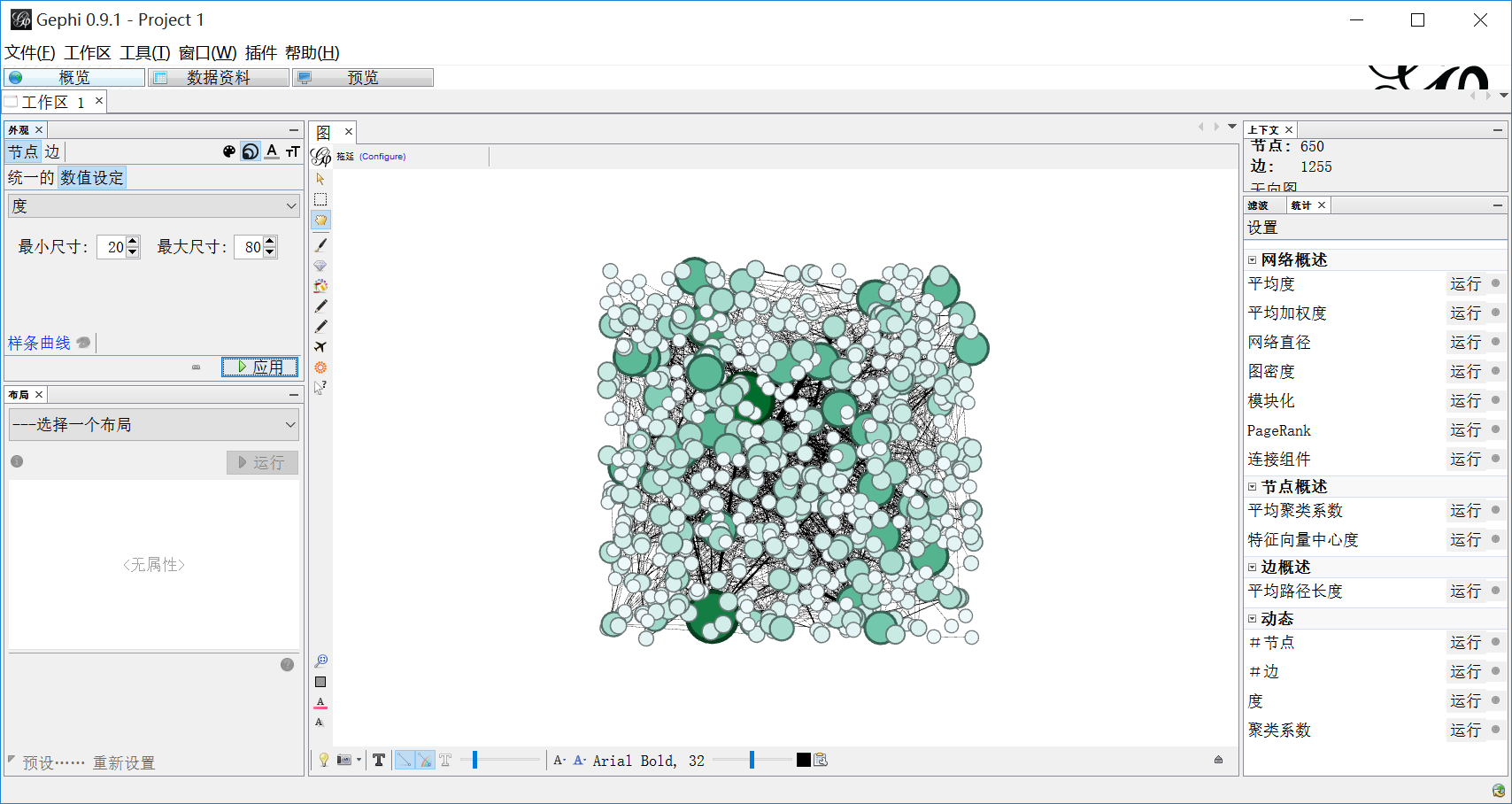2.设置边大小和颜色。
设置边颜色的数值设定为“边的权重”，如下图所示。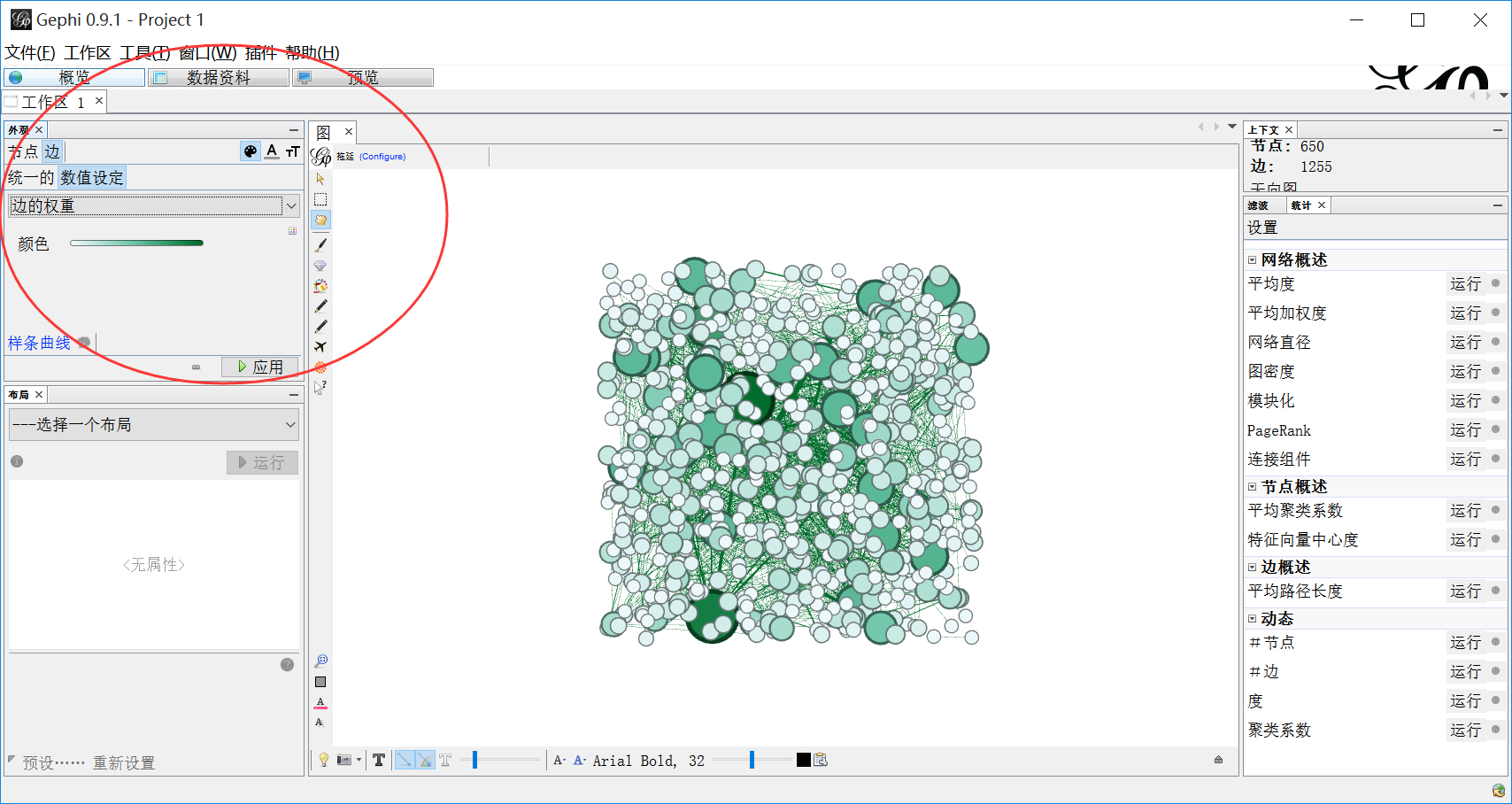设置边的大小数值设定为“边的权重”。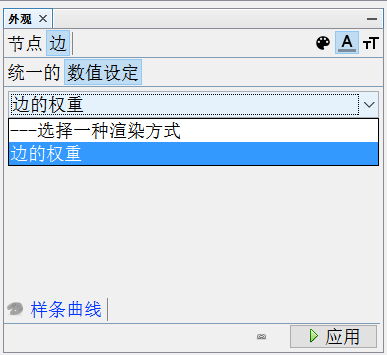3.在布局中选择“Fruchterman Reingold”。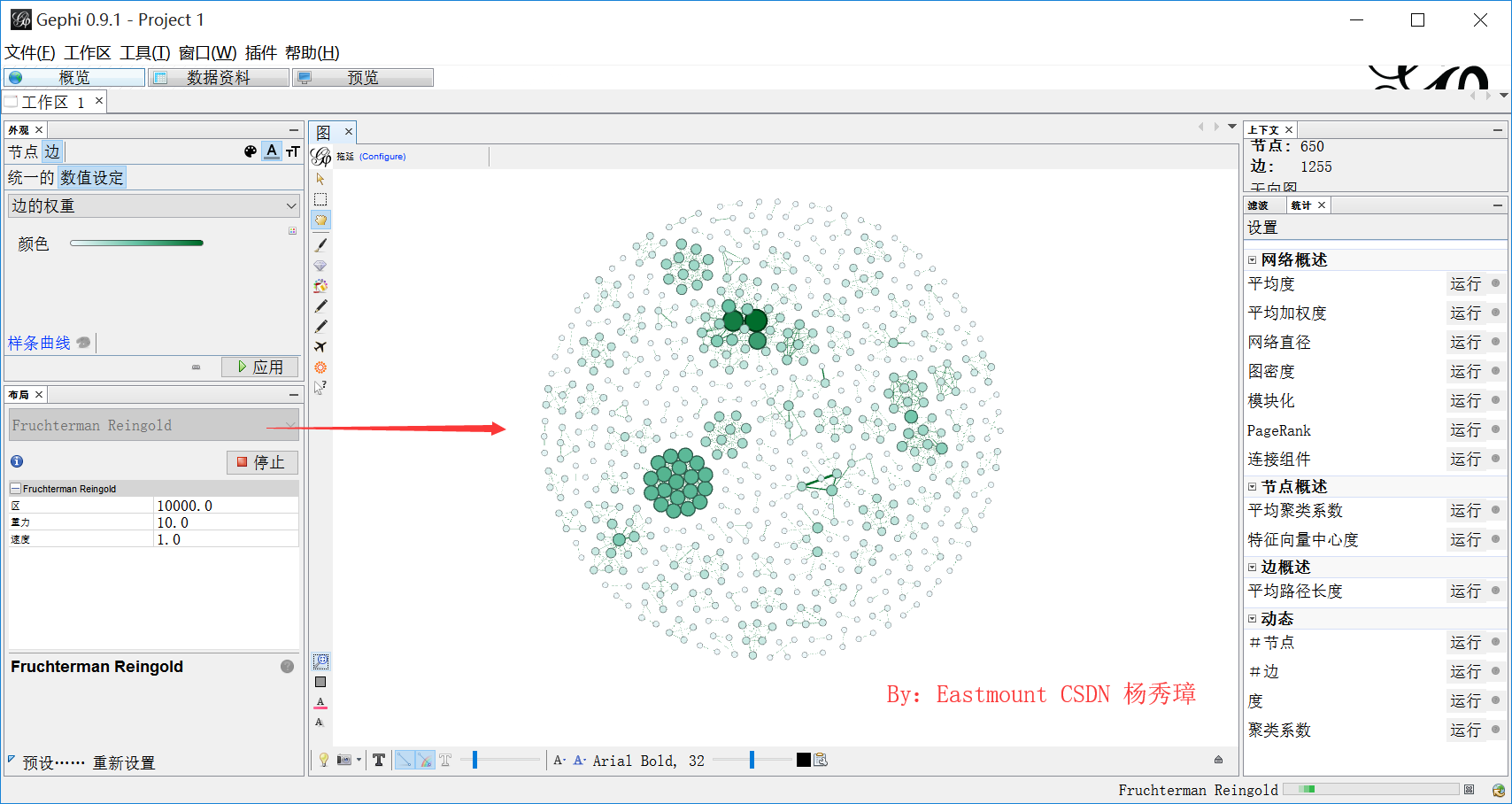显示结果如下所示：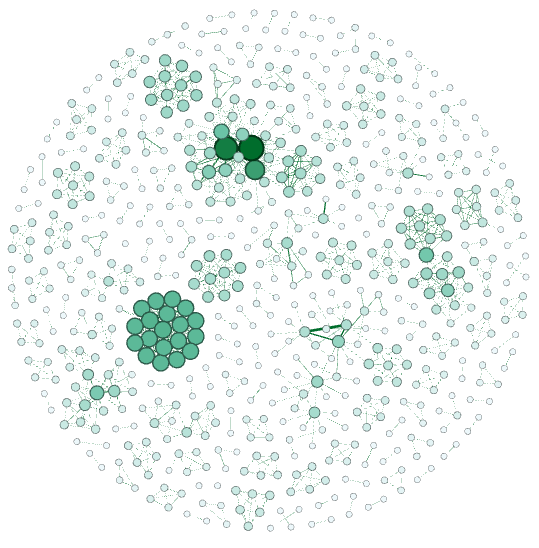4.设置模块化。在右边统计中点击“运行”，设置模块性。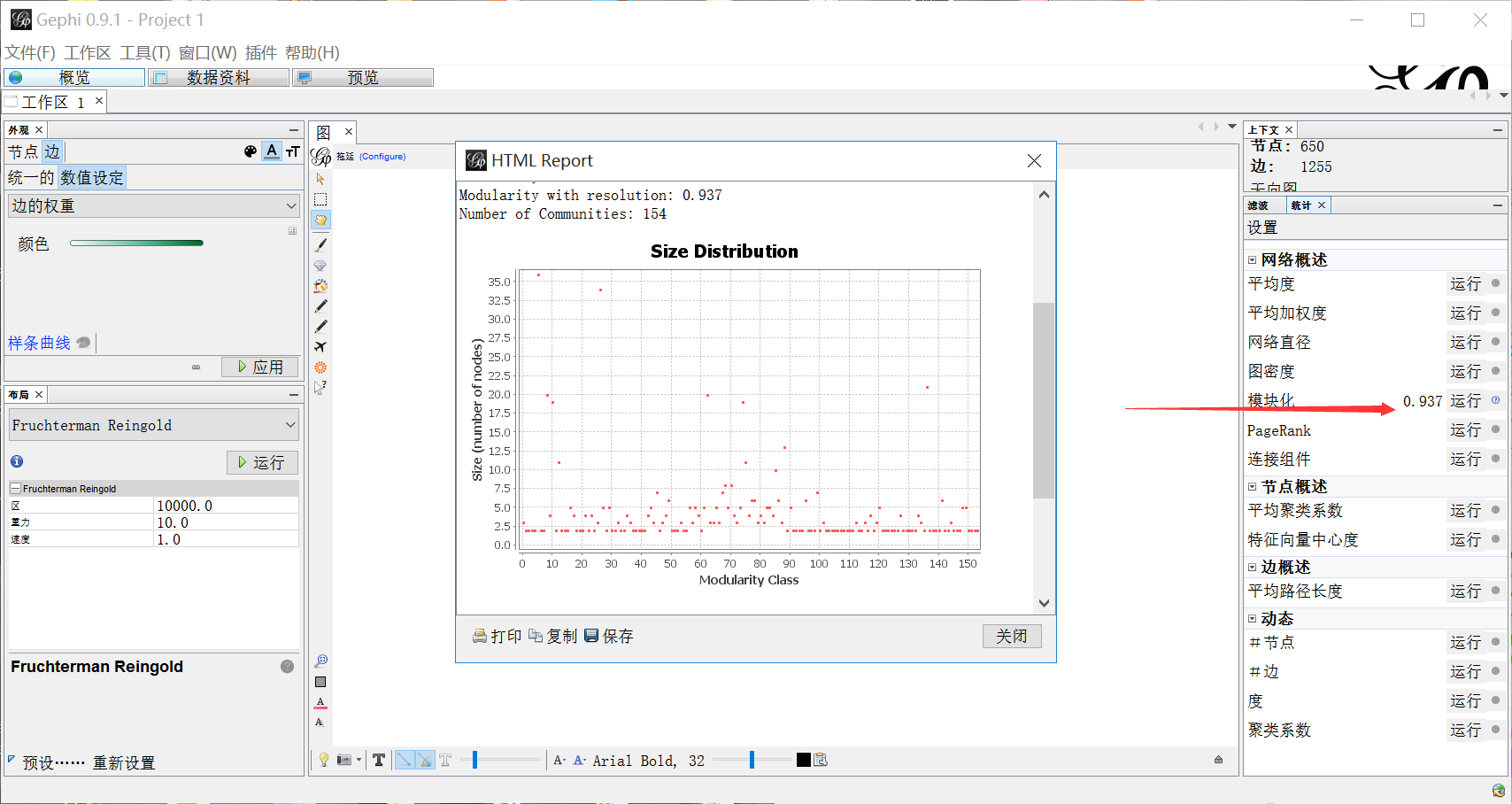5.设置平均路径长度。在右边统计中点击“运行”，设置边概述。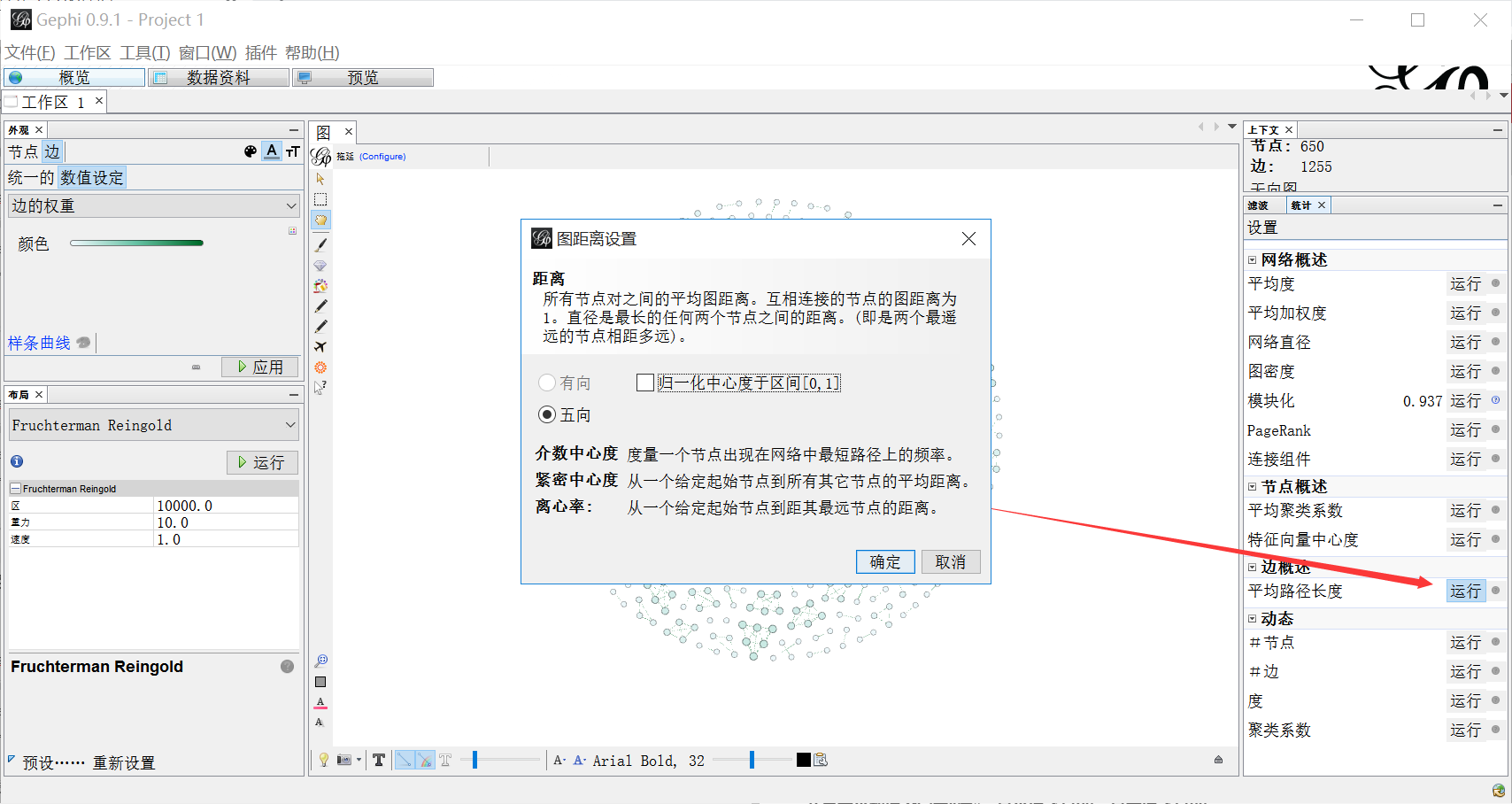6.重新设置节点属性。
节点大小数值设定为“度”，最小值还是20，最大值还是80。
节点颜色数值设定为“Modularity Class”，表示模块化。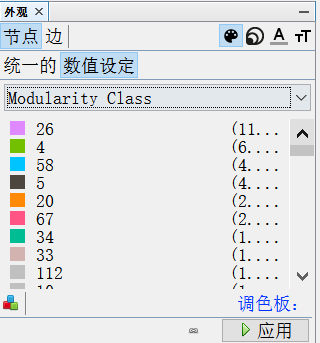此时图形显示如下所示，非常好看，有关系的人物颜色也设置为了类似的颜色。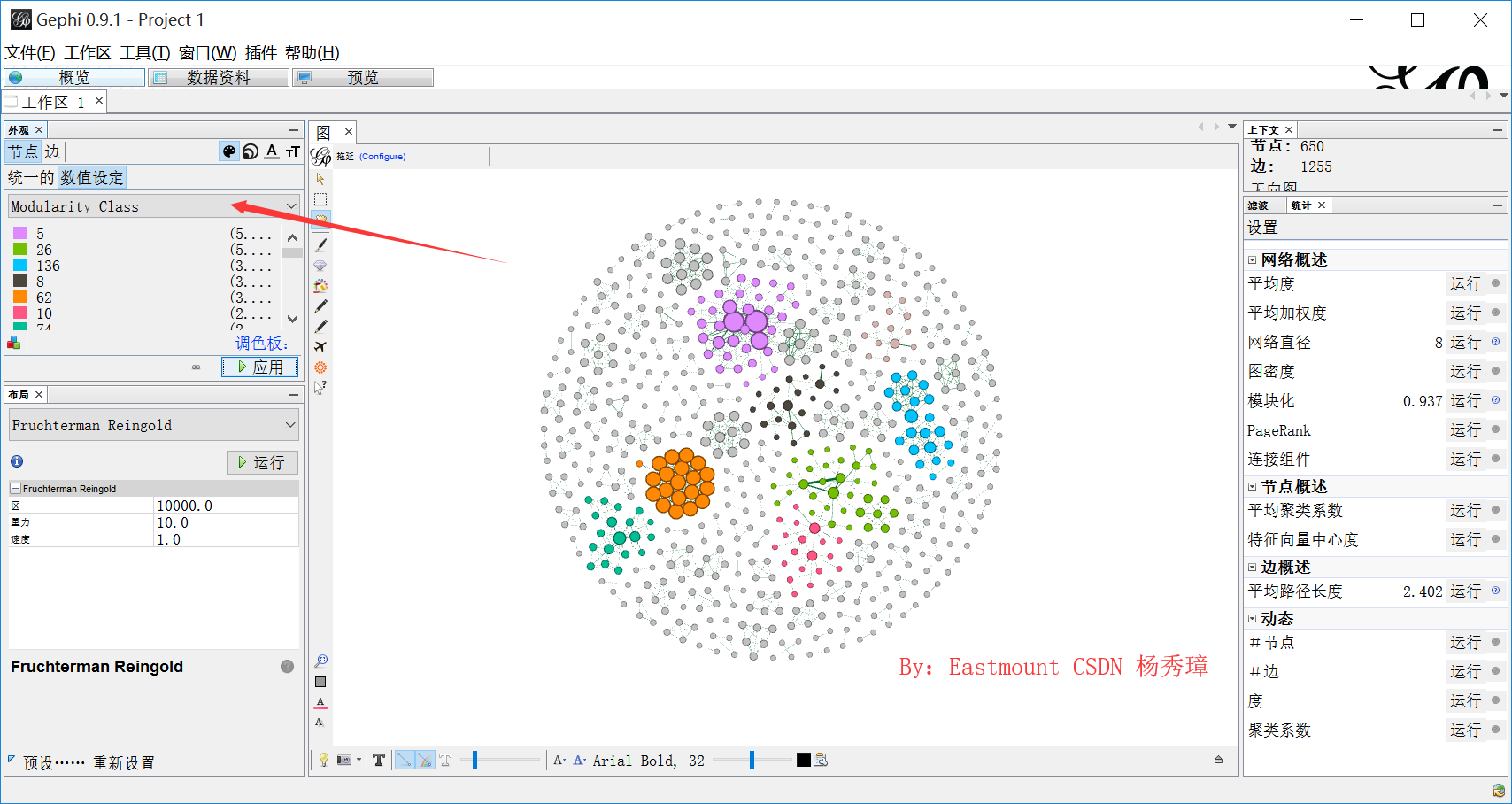(四).可视化显示
显示标签如下图所示：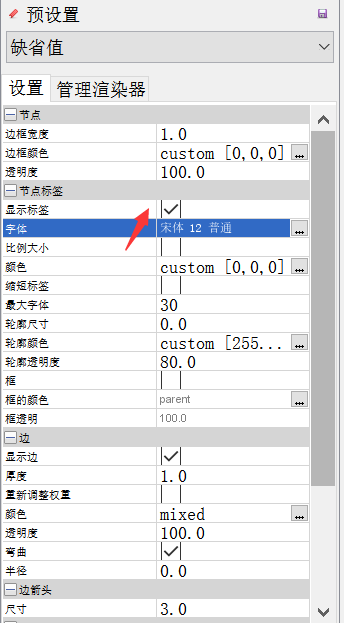最终显示如下图所示。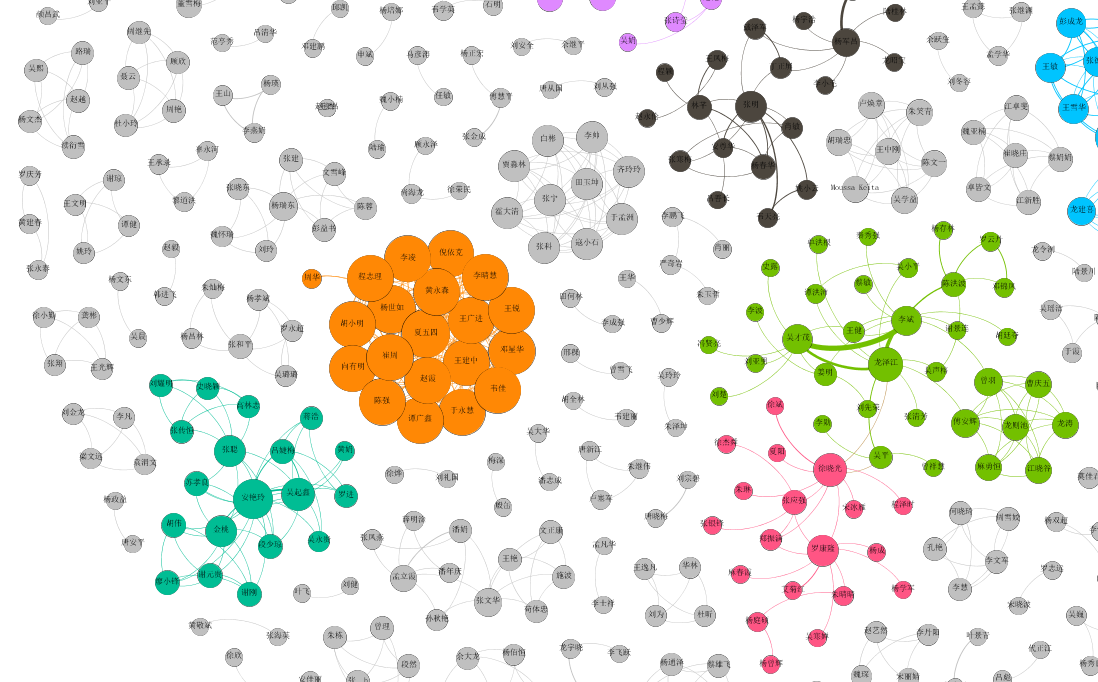讲到这里，整篇文章就结束了，同理可以生成关键词的共现图谱。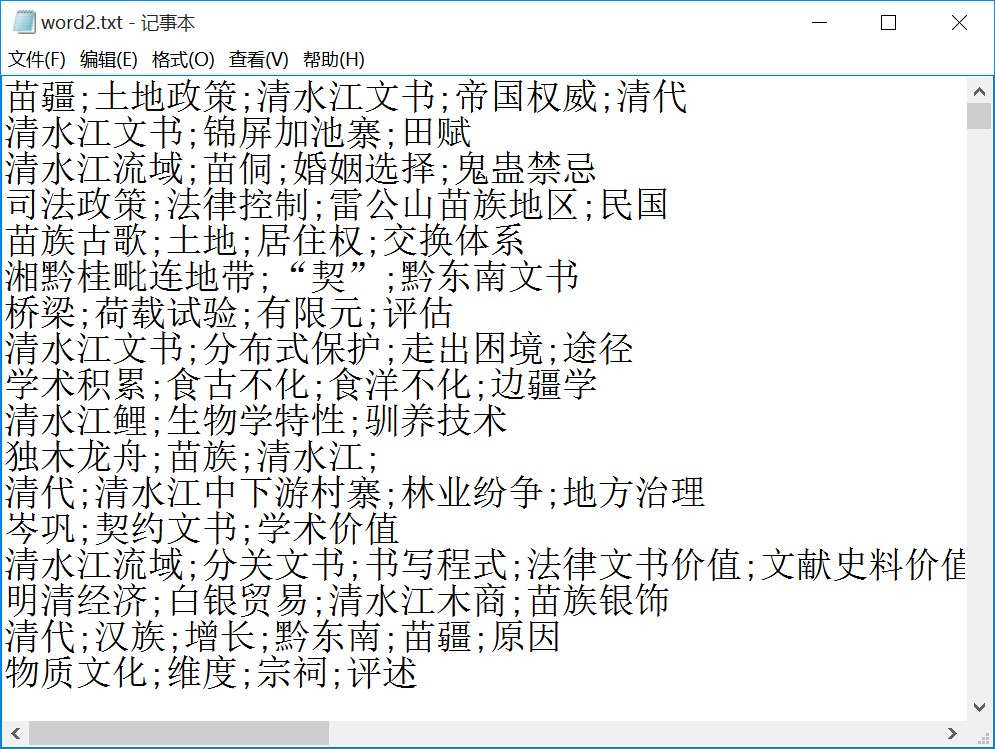同时，如果读者对如何实现以某一个作者为中心的区域领域，后面作者可以再分享一篇文章。

六.总结
最后简单总结下，Neo4图数据库的j缺点是无法设置节点的权重大小，所有节点大小一致，并且运行时间较长。Gephi缺点是不支持语句查询，希望未来自己能搞一个开源的、灵活的关系图谱绘制框架。同时，真正的知识图谱是涉及语义、命名实体识别、关系抽取、实体对齐及消歧等，后面作者在有时间的情况下将继续为读者分享相关知识，前行路上与你同在。
珞珞如石，温而若珈；潇洒坦荡，一生澄澈。新学期，新生活，新里程。
一个多月的闭关，深深体会到数学和英语的重要性，短板抓紧补起来，长板却没有。开学八门课，《信息论》《现代密码学》《网络安全协议》《信息安全前沿》《信息安全与可信计算》《自然语言处理》等，就NLP熟悉点，得加油了，更要静下心来去“做”、“学”、“问”。Stay hungry，Stay foolish~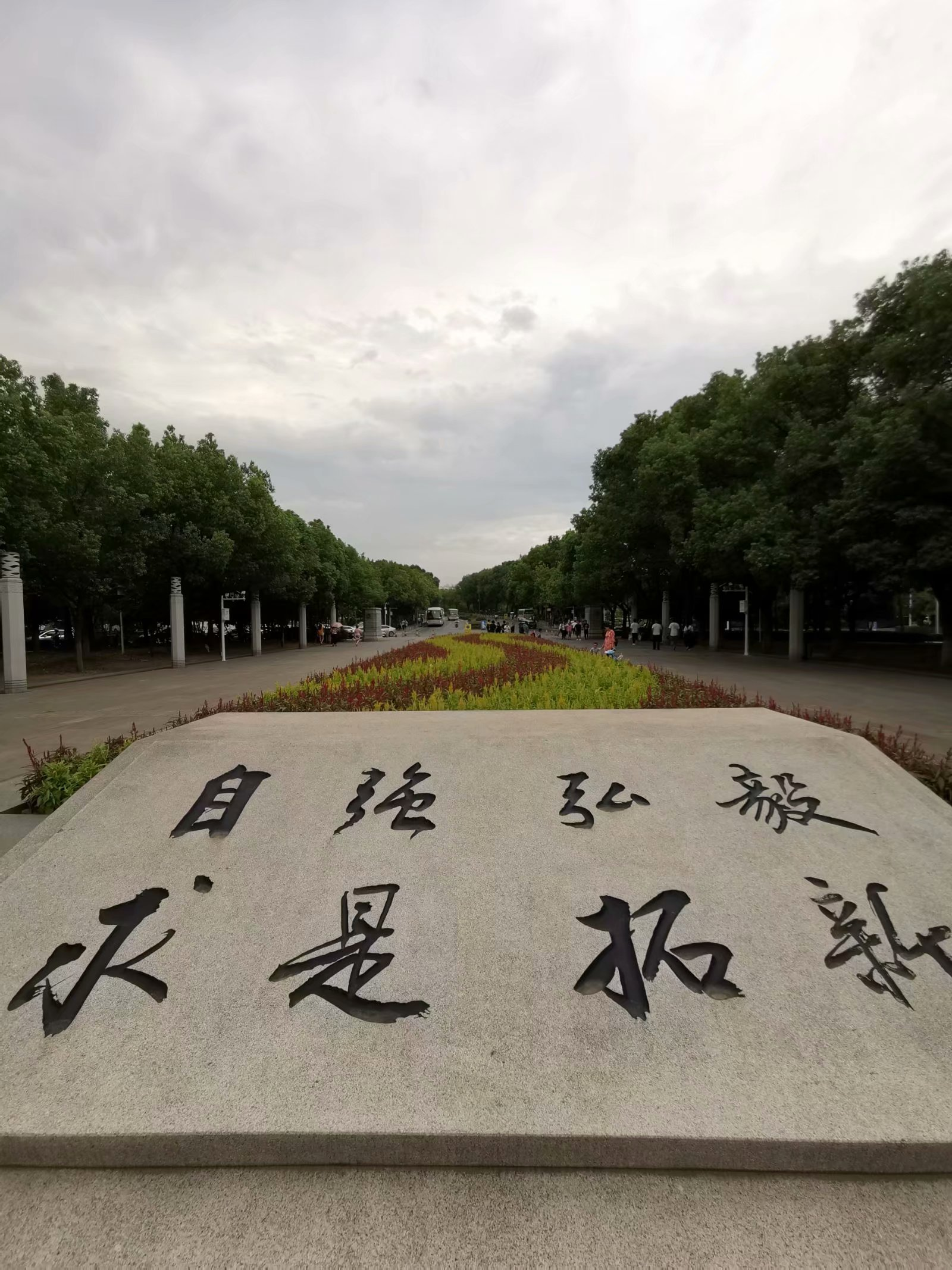(By:Eastmount 2019-09-02 下午5点 http://blog.csdn.net/eastmount/ )


展开全文Gephi 中国知网
• 大家如果对python操作Neo4j导入CSV并不是很熟悉的话...这是本次构建知识图谱的CSV格式的数据源，这是在上次数据的基础上截取了一小部分并且对数据的内容进行了一定的修改便于大家看的更加明显（图中数据信息均为乱改 不
大家如果对python操作Neo4j导入CSV并不是很熟悉的话，可以先看看这篇文章有个大概的操作基础。传送门
大家看过上篇文章应该知道，我们最后构建出来的知识如下图所示，但很明显可以看出来华南师范大学这个节点应该只有一个，应该让所有属于华南师范大学的人物都连接在唯一的节点上而不是每个人物都有属于它自己的节点。这样才会使得我们的图谱更加的紧凑。数据源
这是本次构建知识图谱的CSV格式的数据源，这是在上次数据的基础上截取了一小部分并且对数据的内容进行了一定的修改便于大家看的更加明显（图中数据信息均为乱改 不做实际参考）我们导入CSV格式的文件数据和上次讲的一样，直接读取数据并构建其节点。
with open('D:\\Python\\neo4j-community-4.1.1\\import\\data.csv', 'r',encoding='utf-8') as f:
reader = csv.reader(f)
data = list(reader)
for i  in  range(1,len(data)):
node = Node('person',name = data[i],level= data[i],major = data[i],univer = data[i])
relation = Node('major',name = data[i])
relation1 = Node('univer',name = data[i])
relation2 = Node('level',name = data[i])
graph.create(node)
graph.create(relation)
graph.create(relation1)
graph.create(relation2)

这样构建出来的节点肯定有重复的，比如level这一列我们构建了十个节点但是不重复的节点只有三个，所以我们要把重复的节点都给删除掉。
graph.run('MATCH (n:level) WITH n.name AS name, COLLECT(n) AS nodelist, COUNT(*) AS count WHERE count > 1 CALL apoc.refactor.mergeNodes(nodelist) YIELD node RETURN node')

运行这行程序就可以把level中重复的节点删除掉。其他有重复的也是如此（graph.run()里面的代码也可以在neo4j中运行防止因为python代码出错而显示不出来）
当我们把重复的节点删除以后我们就可以根据关系把节点联系在一起
graph.run('MATCH (entity1:person) , (entity2:major{name:entity1.major}) CREATE (entity1)-[:研究]->(entity2)')
graph.run('MATCH (entity1:person) , (entity2:univer{name:entity1.univer}) CREATE (entity1)-[:学校]->(entity2)')
graph.run('MATCH (entity1:person) , (entity2:level{name:entity1.level}) CREATE (entity1)-[:学位]->(entity2)')

最后运行出来 结果如下：基于python的Django框架和Neo4j的知识图谱可视化


展开全文neo4j
• 基于python+neo4j的豆瓣电影知识图谱，与cypher查询语句相关，主要是爬虫代码及数据，有意向者可下载
• 1. 首先对网页数据进行分析，进而确定节点和关系 我们直接分析电影点进去的详细页面，页面如下：（由于豆瓣在没有登录的情况下频繁对网站进行请求会被认为恶意攻击，导致自己的ip无法访问该网站，所以最好先下载...neo4j
•  利用Gephi构建知识图谱，无外乎两点：节点信息和边界信息。节点数据还是很好处理的，将金庸武侠世界的所有有名有姓的人物取为节点数据即可，关键在于边界数据的提取。参考众多大神的经验，大部分是...Gephi
• https://zhuanlan.zhihu.com/p/243211697
• 2、Python 连接 Neo4j 图数据库 2.1、安装 Python 所需的连接驱动 pip install py2neo 可能会有安装包的依赖，因为是在 Anaconda 上拓展包，依赖包一般都有，如果有需要安装的提示，按照提示安装即可。 2.2、创建...图数据库
• 小型金融知识图谱构建流程 小型金融知识图谱构流程示范 小型金融知识图谱构流程示范 1 知识图谱存储方式 2 图数据库neo4j 2.1 下载 2.2 启动 2.2.1 打开 http://localhost:7474 2.2.2 初始账户和密码均为neo4j......

# python构建知识图谱python 订阅